Below you’ll find the complete code used to create the ggplot2 graphs in my talk The Good, the Bad and the Ugly: how (not) to visualize data at this year’s data2day conference. You can find the German slides here:

You can also find a German blog article accompanying my talk on codecentric’s blog.

If you have questions or would like to talk about this article (or something else data-related), you can now book 15-minute timeslots with me (it’s free - one slot available per weekday):If you have been enjoying my content and would like to help me be able to create more, please consider sending me a donation at . Thank you! :-)

``````library(tidyverse)

library(ggExtra)
library(ragg)
library(ggalluvial)
library(treemapify)
library(ggalt)

library(palmerpenguins)``````

## Dataset

``head(penguins)``
``````## # A tibble: 6 x 8
##   species island bill_length_mm bill_depth_mm flipper_length_… body_mass_g sex
##   <fct>   <fct>           <dbl>         <dbl>            <int>       <int> <fct>
## 1 Adelie  Torge…           39.1          18.7              181        3750 male
## 2 Adelie  Torge…           39.5          17.4              186        3800 fema…
## 3 Adelie  Torge…           40.3          18                195        3250 fema…
## 4 Adelie  Torge…           NA            NA                 NA          NA <NA>
## 5 Adelie  Torge…           36.7          19.3              193        3450 fema…
## 6 Adelie  Torge…           39.3          20.6              190        3650 male
## # … with 1 more variable: year <int>``````
``#head(penguins_raw)``

## Colors

``````# The palette with grey:
cbp1 <- c("#999999", "#E69F00", "#56B4E9", "#009E73",
"#F0E442", "#0072B2", "#D55E00", "#CC79A7")

# The palette with black:
cbp2 <- c("#000000", "#E69F00", "#56B4E9", "#009E73",
"#F0E442", "#0072B2", "#D55E00", "#CC79A7")

library(plotrix)
sliceValues <- rep(10, 8) # each slice value=10 for proportionate slices
(
p <- pie3D(sliceValues,
explode=0,
theta = 1.2,
col = cbp1,
labels = cbp1,
labelcex = 0.9,
main = "Colorblind\nfriendly palette")
)````````````##  0.3926991 1.1780972 1.9634954 2.7488936 3.5342917 4.3196899 5.1050881
##  5.8904862``````
``````ggplot <- function(...) ggplot2::ggplot(...) +
scale_color_manual(values = cbp1) +
scale_fill_manual(values = cbp1) + # note: needs to be overridden when using continuous color scales
theme_bw()``````

## Main diagram types

### Pointcharts

``````penguins %>%
remove_missing() %>%
ggplot(aes(x = bill_length_mm, y = flipper_length_mm)) +
geom_jitter(alpha = 0.5) +
facet_wrap(vars(species), ncol = 3) +
scale_x_reverse() +
scale_y_reverse() +
labs(x = "Bill length (mm)",
y = "Flipper length (mm)",
size = "body mass (g)",
title = "Scatterplot",
subtitle = "Penguins bill v. flipper length by species",
caption = "Source: https://github.com/allisonhorst/palmerpenguins")````````````penguins %>%
remove_missing() %>%
ggplot(aes(x = bill_length_mm, y = flipper_length_mm,
color = species, shape = species)) +
geom_point(alpha = 0.7) +
labs(x = "Bill length (mm)",
y = "Flipper length (mm)",
title = "Scatterplot",
subtitle = "Penguins bill v. flipper length by species",
caption = "Source: https://github.com/allisonhorst/palmerpenguins")``````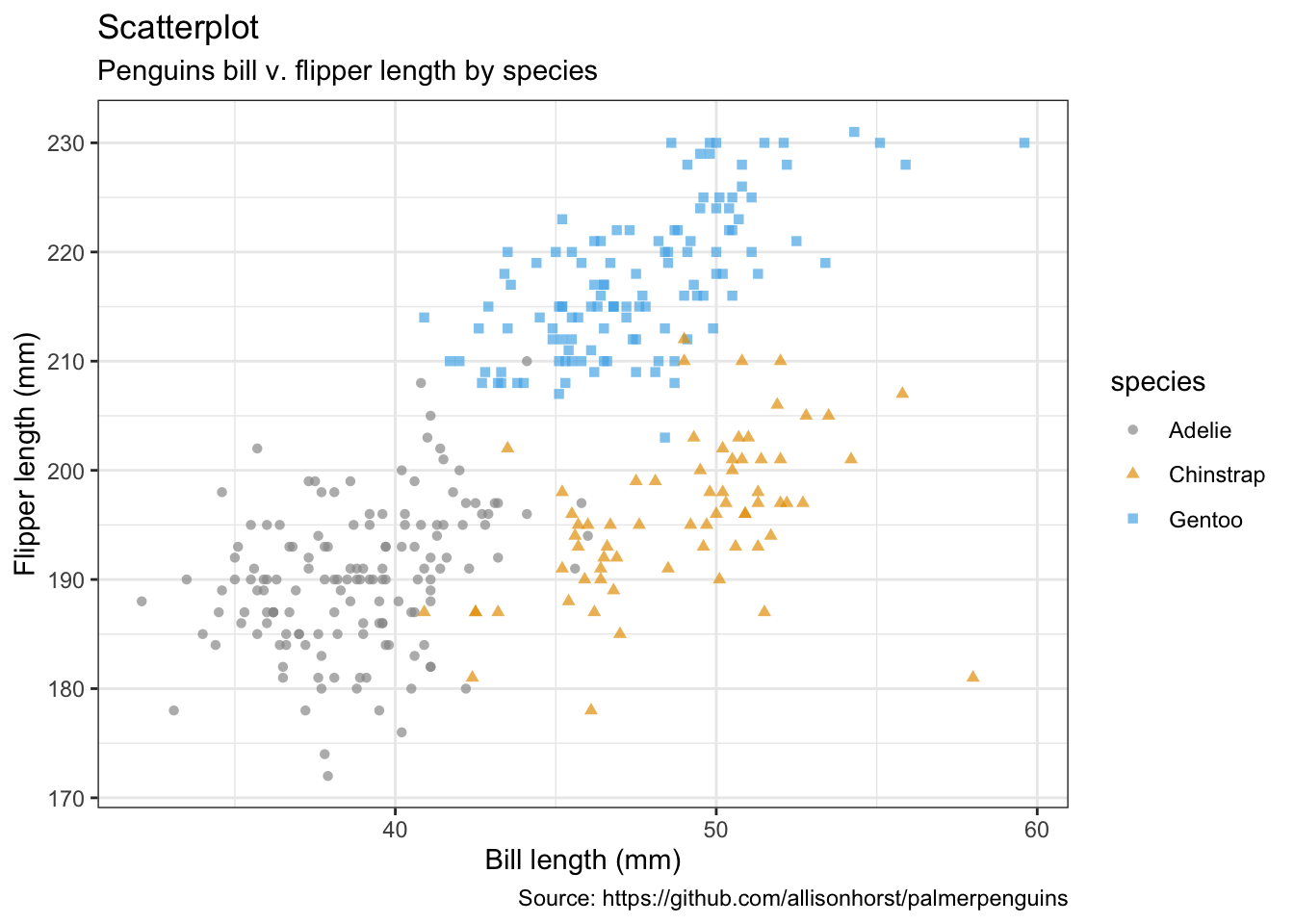• Jitter with smoothing line
``````penguins %>%
remove_missing() %>%
ggplot(aes(x = bill_length_mm, y = flipper_length_mm,
color = species, shape = species)) +
geom_jitter(alpha = 0.5) +
geom_smooth(method = "loess", se = TRUE) +
facet_wrap(vars(species), nrow = 3) +
labs(x = "Bill length (mm)",
y = "Flipper length (mm)",
title = "Scatterplot with smoothing line",
subtitle = "Penguins bill v. flipper length by species with loess smoothing line",
caption = "Source: https://github.com/allisonhorst/palmerpenguins")````````````penguins %>%
remove_missing() %>%
ggplot(aes(x = bill_length_mm, y = flipper_length_mm)) +
geom_point(alpha = 0.5) +
geom_smooth(method = "loess", se = TRUE) +
labs(x = "Bill length (mm)",
y = "Flipper length (mm)",
title = "Scatterplot with smoothing line",
subtitle = "Penguins bill v. flipper length by species with\nloess smoothing line, histogram & density distribution",
caption = "Source: https://github.com/allisonhorst/palmerpenguins")``````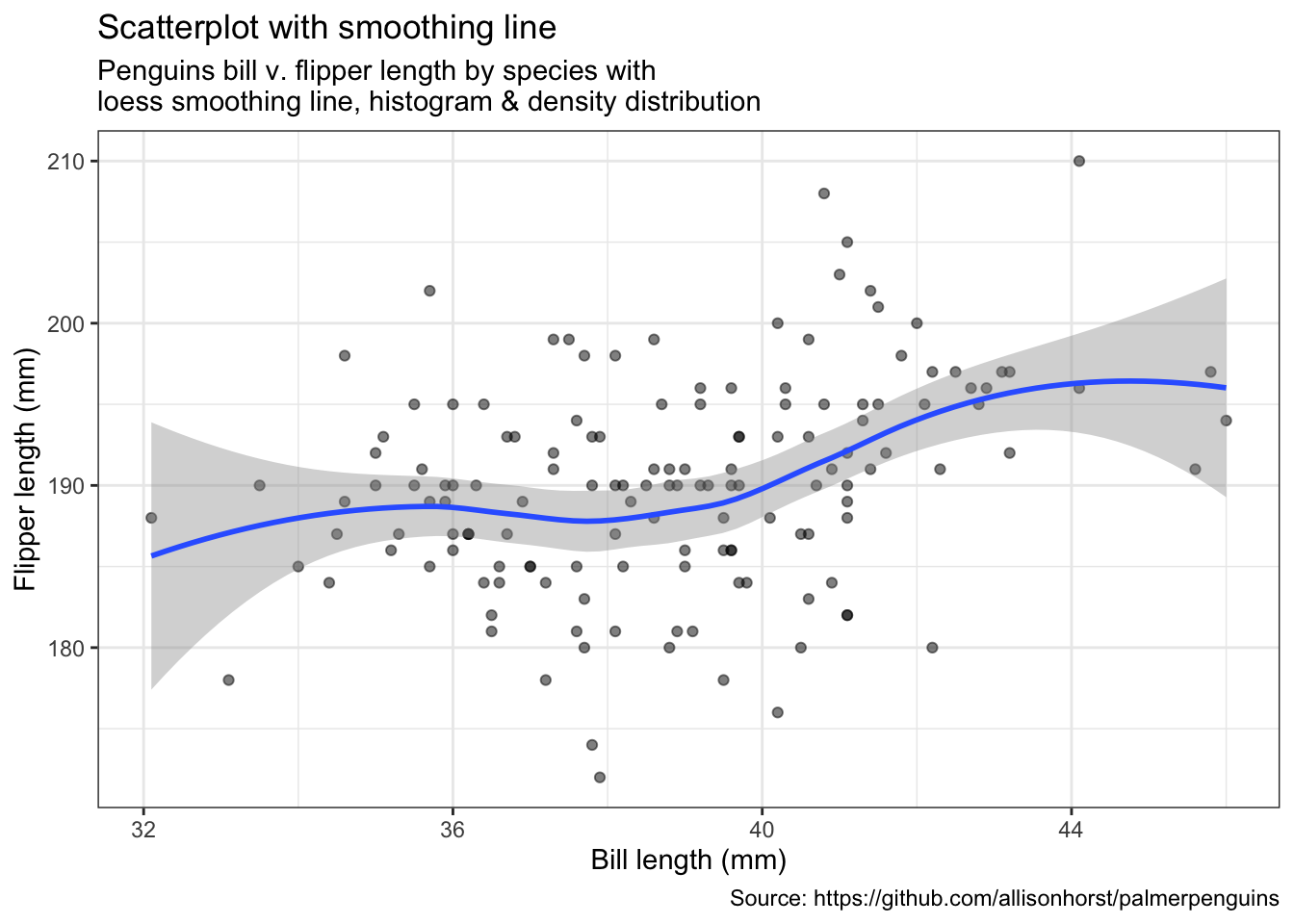``#(ggMarginal(p, type = "densigram", fill = "transparent"))``

### Bubblecharts

``````penguins %>%
remove_missing() %>%
ggplot(aes(x = bill_length_mm, y = flipper_length_mm,
color = species, shape = species, size = body_mass_g)) +
geom_point(alpha = 0.5) +
labs(x = "Bill length (mm)",
y = "Flipper length (mm)",
title = "Bubble plot",
size = "body mass (g)",
subtitle = "Penguins bill v. flipper length by species;\nsize indicates body mass in grams",
caption = "Source: https://github.com/allisonhorst/palmerpenguins")``````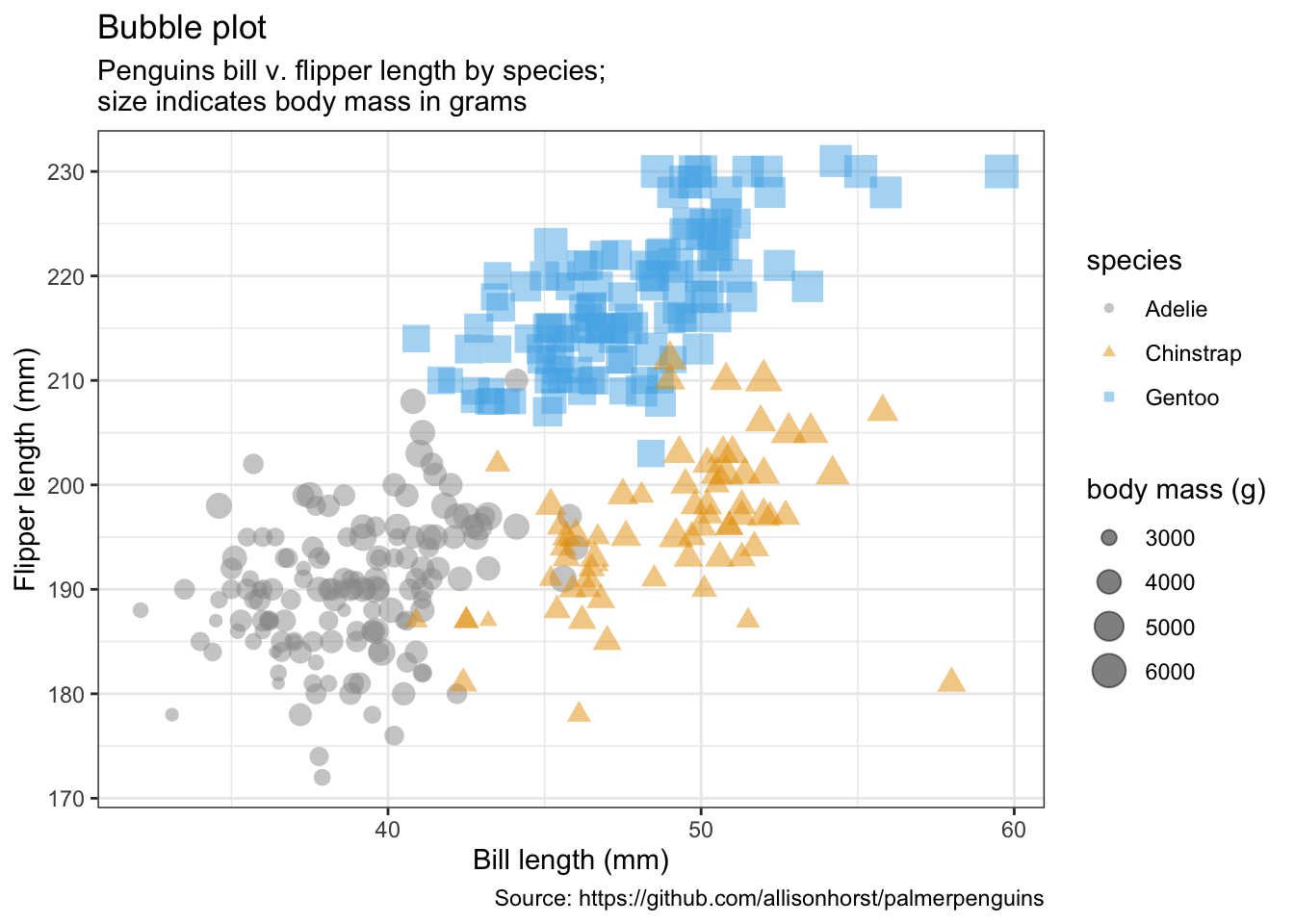### Linecharts

``````penguins %>%
remove_missing() %>%
ggplot(aes(x = bill_length_mm, y = flipper_length_mm,
color = sex)) +
geom_line() +
geom_point() +
labs(x = "Bill length (mm)",
y = "Flipper length (mm)",
title = "Line plot",
subtitle = "Penguins bill v. flipper length by species and sex",
caption = "Source: https://github.com/allisonhorst/palmerpenguins")``````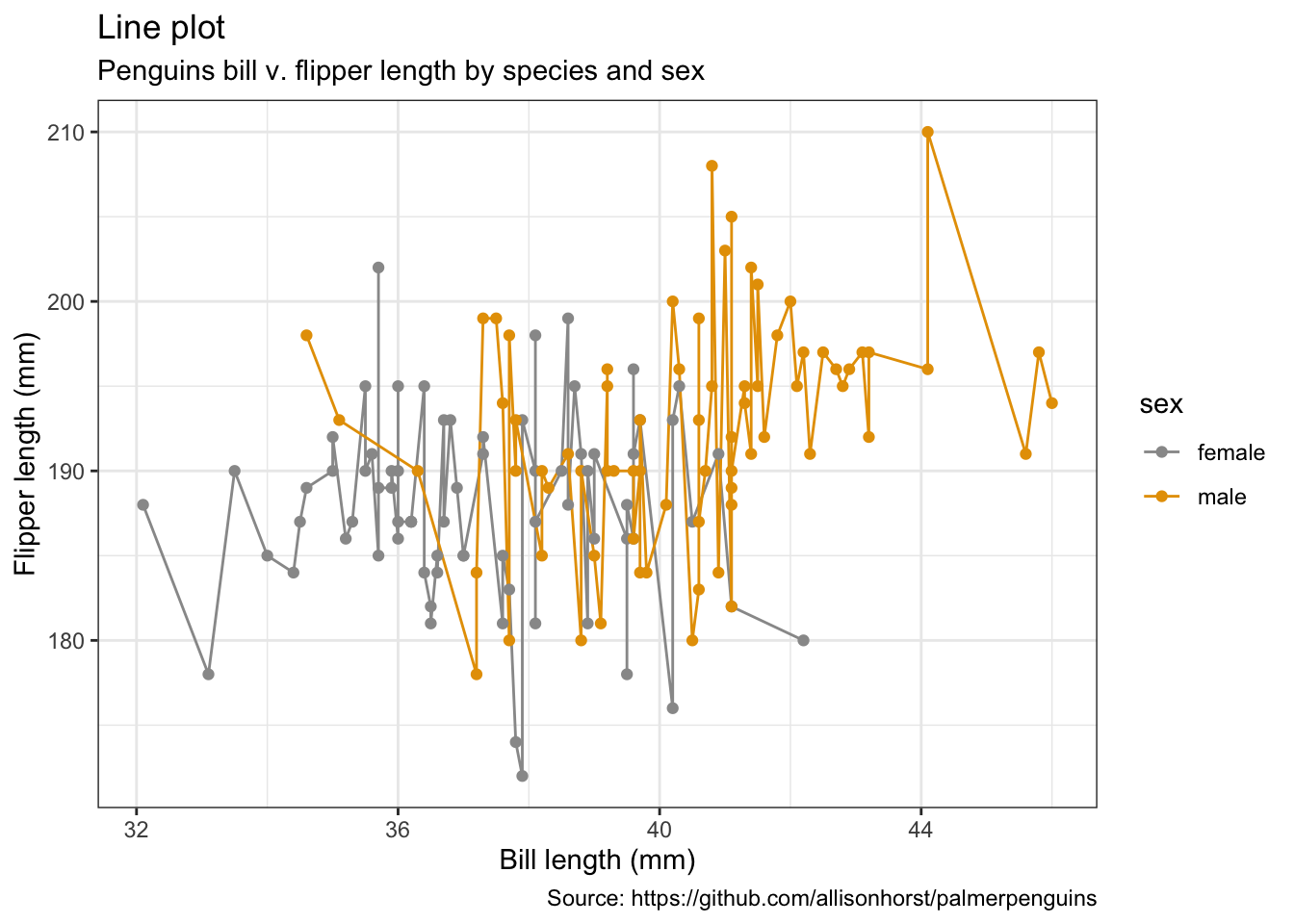### Correlation plots / heatmaps

``````mat <- penguins %>%
remove_missing() %>%
select(bill_depth_mm, bill_length_mm, body_mass_g, flipper_length_mm)

cormat <- round(cor(mat), 2)
cormat[upper.tri(cormat)] <- NA

cormat <- cormat %>%
as_data_frame() %>%
mutate(x = colnames(mat)) %>%
gather(key = "y", value = "value", bill_depth_mm:flipper_length_mm)

cormat %>%
remove_missing() %>%
arrange(x, y) %>%
ggplot(aes(x = x, y = y, fill = value)) +
geom_tile() +
scale_fill_gradient2(low = "blue", high = "red", mid = "white",
midpoint = 0, limit = c(-1,1), space = "Lab",
name = "Pearson\nCorrelation") +
theme(axis.text.x = element_text(angle = 45, vjust = 1, hjust = 1)) +
coord_fixed() +
labs(x = "",
y = "",
title = "Correlation heatmap",
subtitle = "Correlation btw. penguins' traits",
caption = "Source: https://github.com/allisonhorst/palmerpenguins")``````### Barcharts

• per default: counts
``````penguins %>%
remove_missing() %>%
ggplot(aes(x = species,
fill = sex)) +
geom_bar() +
labs(x = "Species",
y = "Counts",
title = "Barchart",
subtitle = "Counts of male & female penguins per species in study",
caption = "Source: https://github.com/allisonhorst/palmerpenguins")``````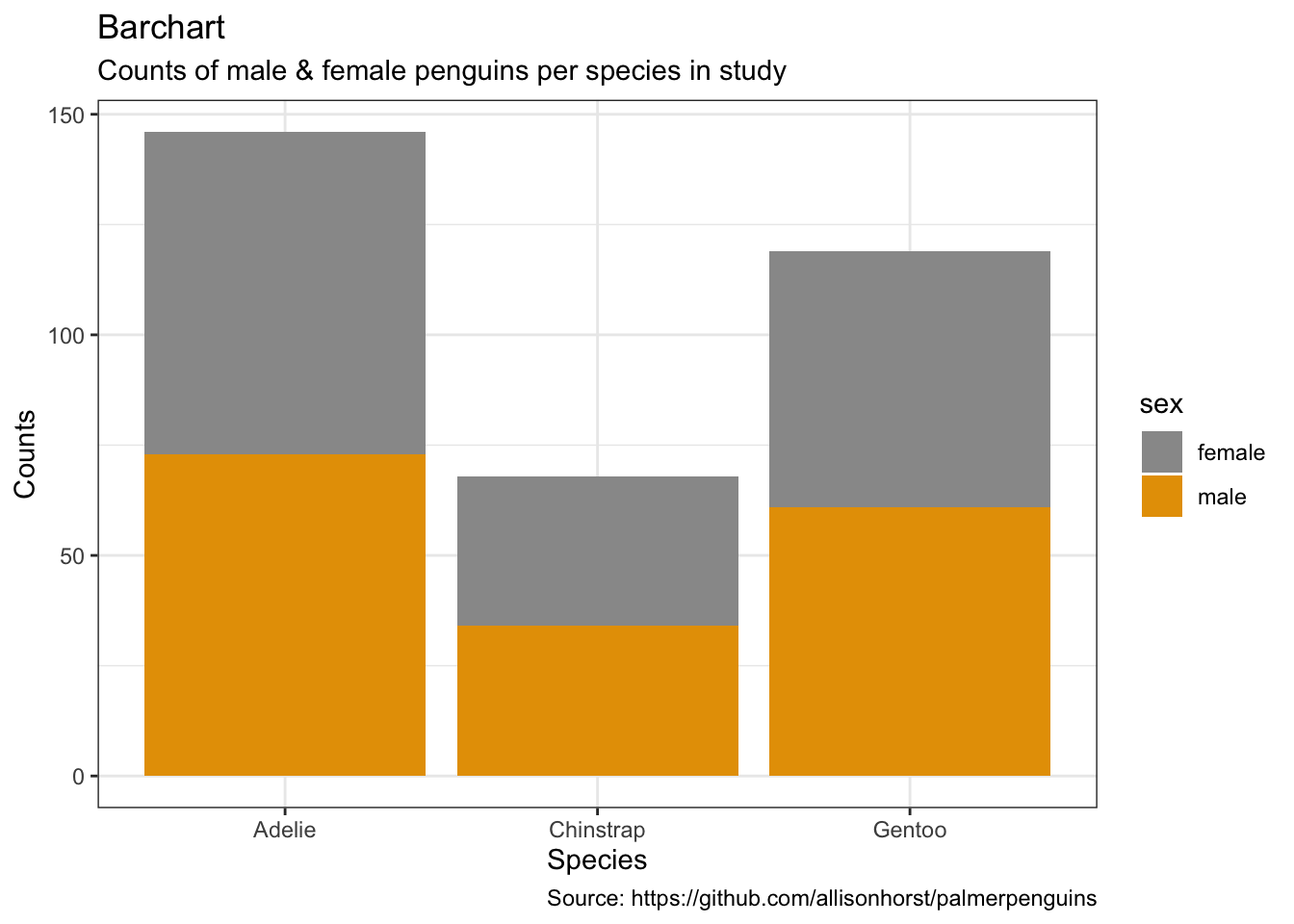``````penguins %>%
remove_missing() %>%
ggplot(aes(x = species,
fill = sex)) +
geom_bar(position = 'dodge') +
labs(x = "Species",
y = "Counts",
title = "Barchart",
subtitle = "Counts of male & female penguins per species in study",
caption = "Source: https://github.com/allisonhorst/palmerpenguins")``````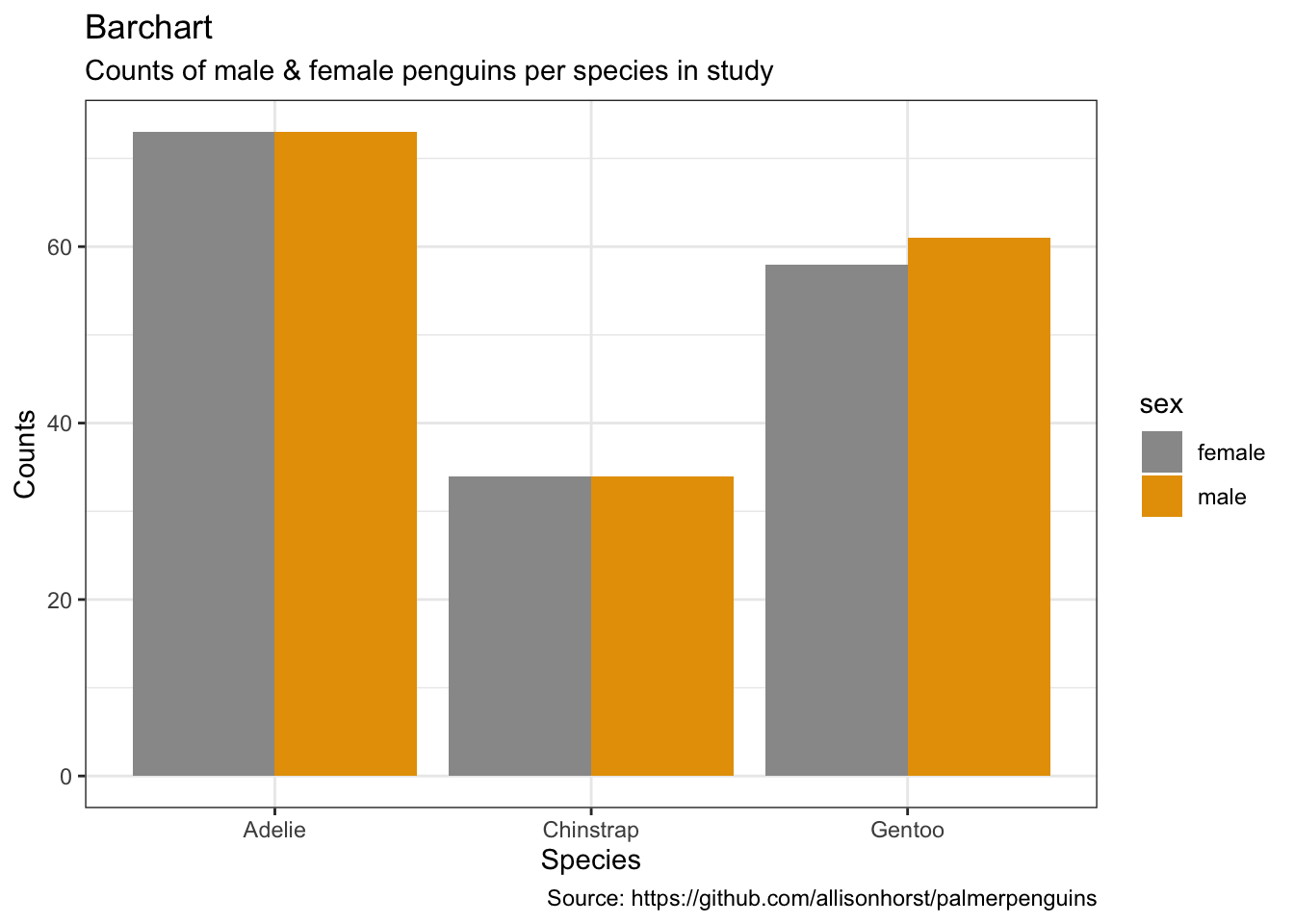• alternative: set y-values
``````penguins %>%
remove_missing() %>%
group_by(species, sex) %>%
summarise(mean_bmg = mean(body_mass_g),
sd_bmg = sd(body_mass_g)) %>%
ggplot(aes(x = species, y = mean_bmg,
fill = sex)) +
geom_bar(stat = "identity", position = "dodge") +
geom_errorbar(aes(ymin = mean_bmg - sd_bmg,
ymax = mean_bmg + sd_bmg),
width = 0.2,
position = position_dodge(0.9)) +
labs(x = "Species",
y = "Mean body mass (in g)",
title = "Barchart",
subtitle = "Mean body mass of male & female penguins per species\nwith standard deviation",
caption = "Source: https://github.com/allisonhorst/palmerpenguins")``````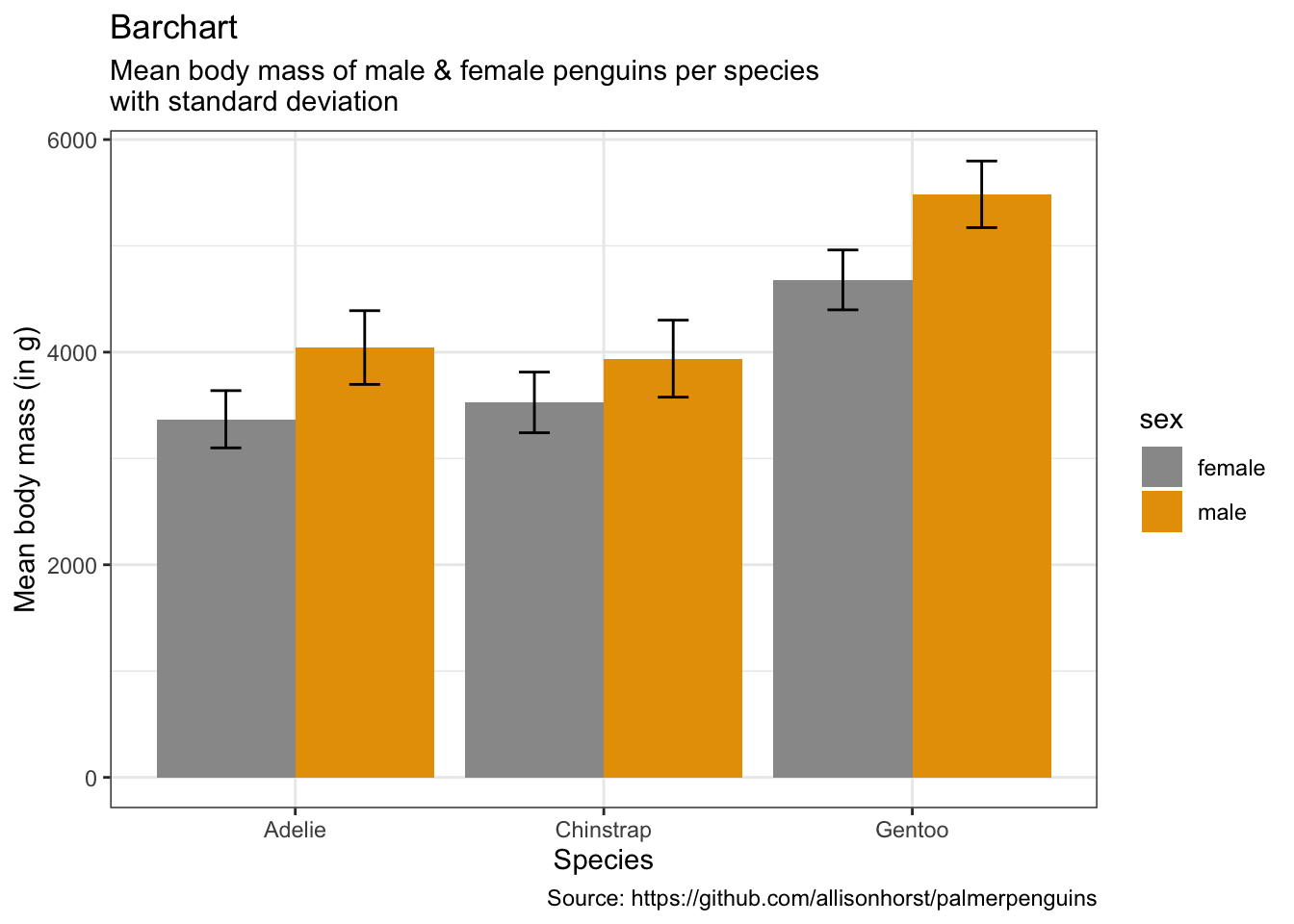### Boxplots

``````penguins %>%
remove_missing() %>%
ggplot(aes(x = species, y = body_mass_g,
fill = sex)) +
geom_boxplot() +
labs(x = "Species",
y = "Body mass (in g)",
title = "Boxplot",
subtitle = "Body mass of three penguin species per sex",
caption = "Source: https://github.com/allisonhorst/palmerpenguins")``````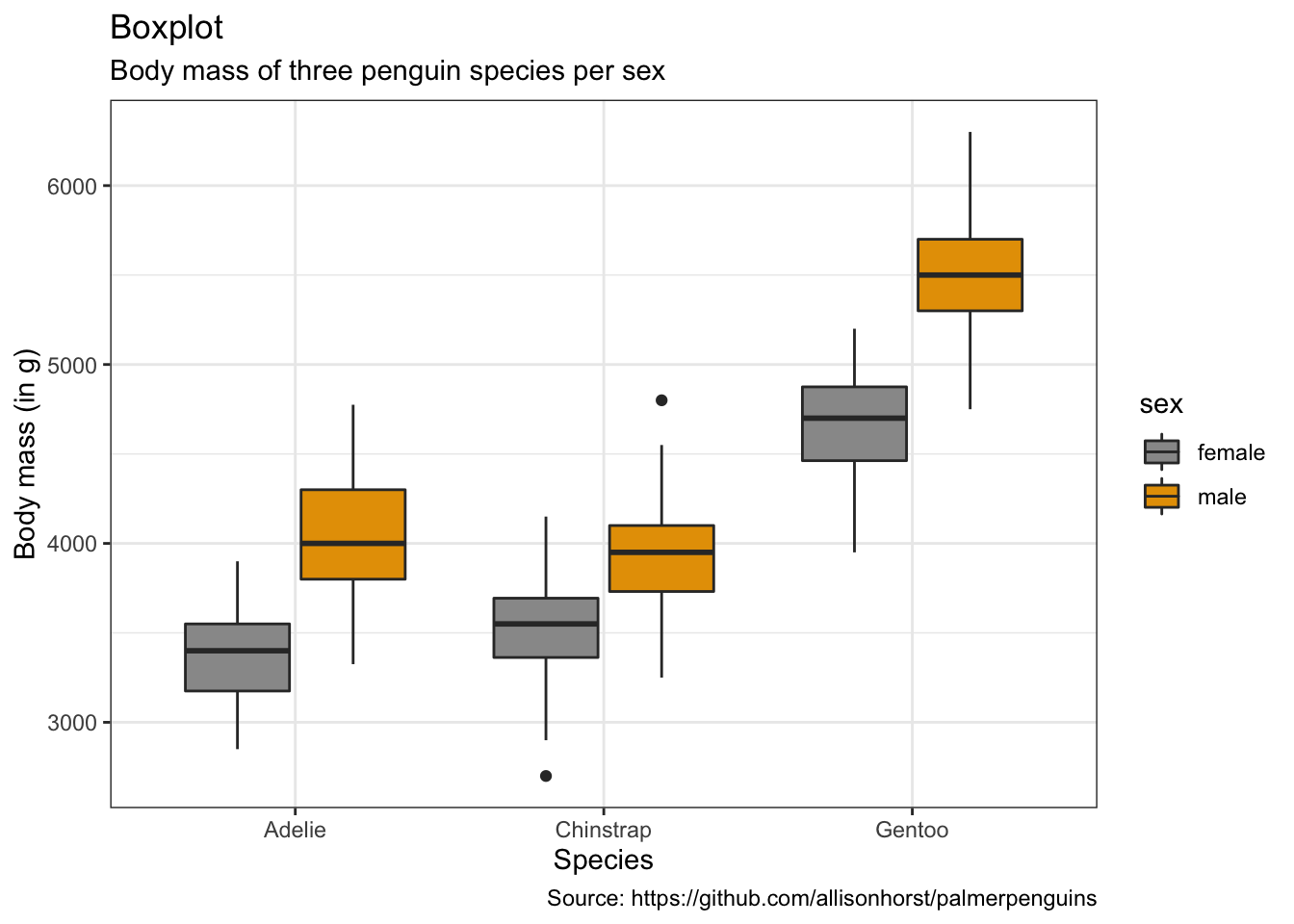• with points
``````penguins %>%
remove_missing() %>%
ggplot(aes(x = species, y = body_mass_g,
fill = sex, color = sex)) +
geom_boxplot(alpha = 0.5, notch = TRUE) +
geom_jitter(alpha = 0.5, position=position_jitter(0.3)) +
labs(x = "Species",
y = "Body mass (in g)",
title = "Boxplot with points (dotplot)",
subtitle = "Body mass of three penguin species per sex",
caption = "Source: https://github.com/allisonhorst/palmerpenguins")``````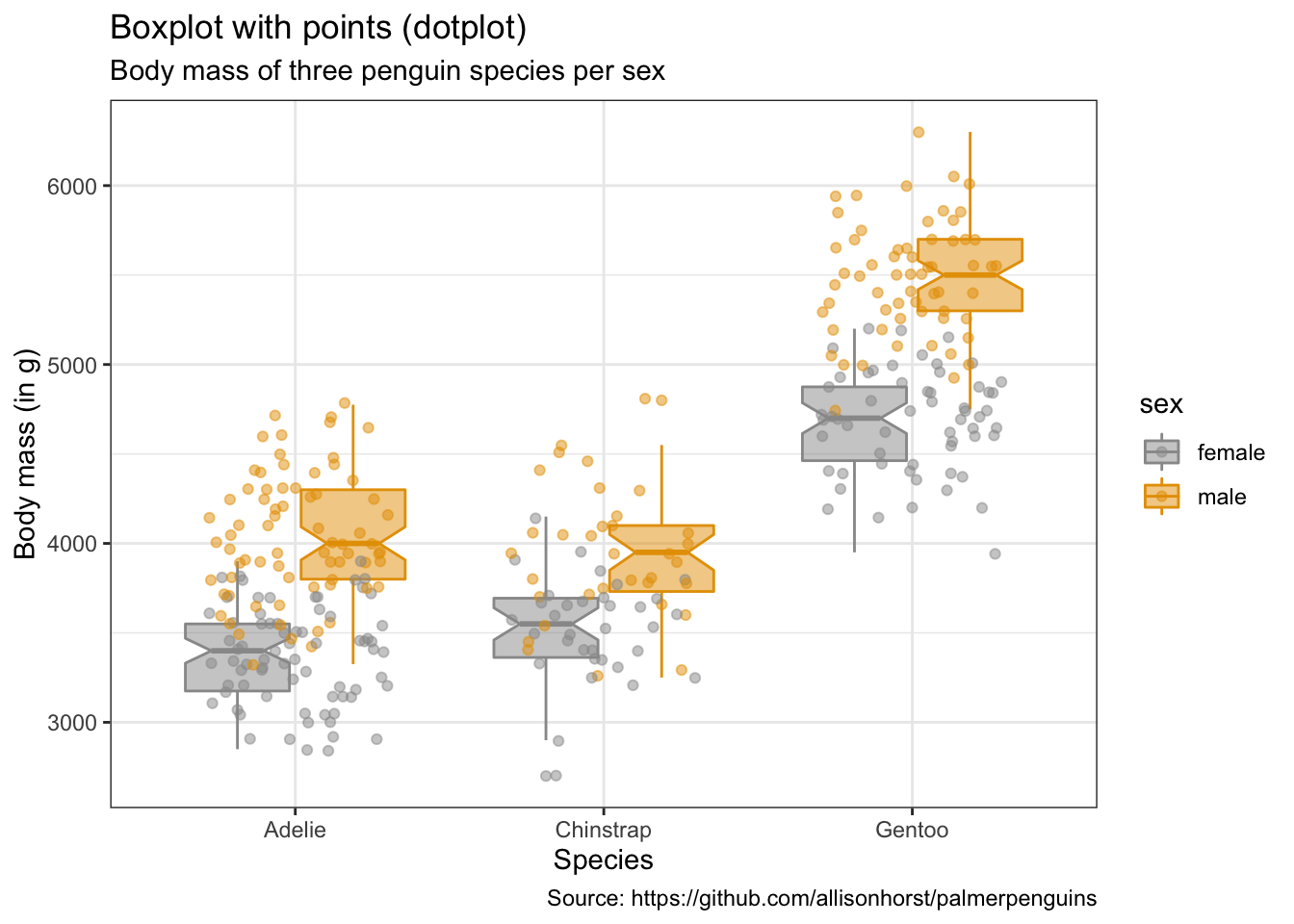### Violinplots

``````penguins %>%
remove_missing() %>%
ggplot(aes(x = species, y = body_mass_g,
fill = sex)) +
geom_violin(scale = "area") +
labs(x = "Species",
y = "Body mass (in g)",
title = "Violinplot",
subtitle = "Body mass of three penguin species per sex",
caption = "Source: https://github.com/allisonhorst/palmerpenguins")``````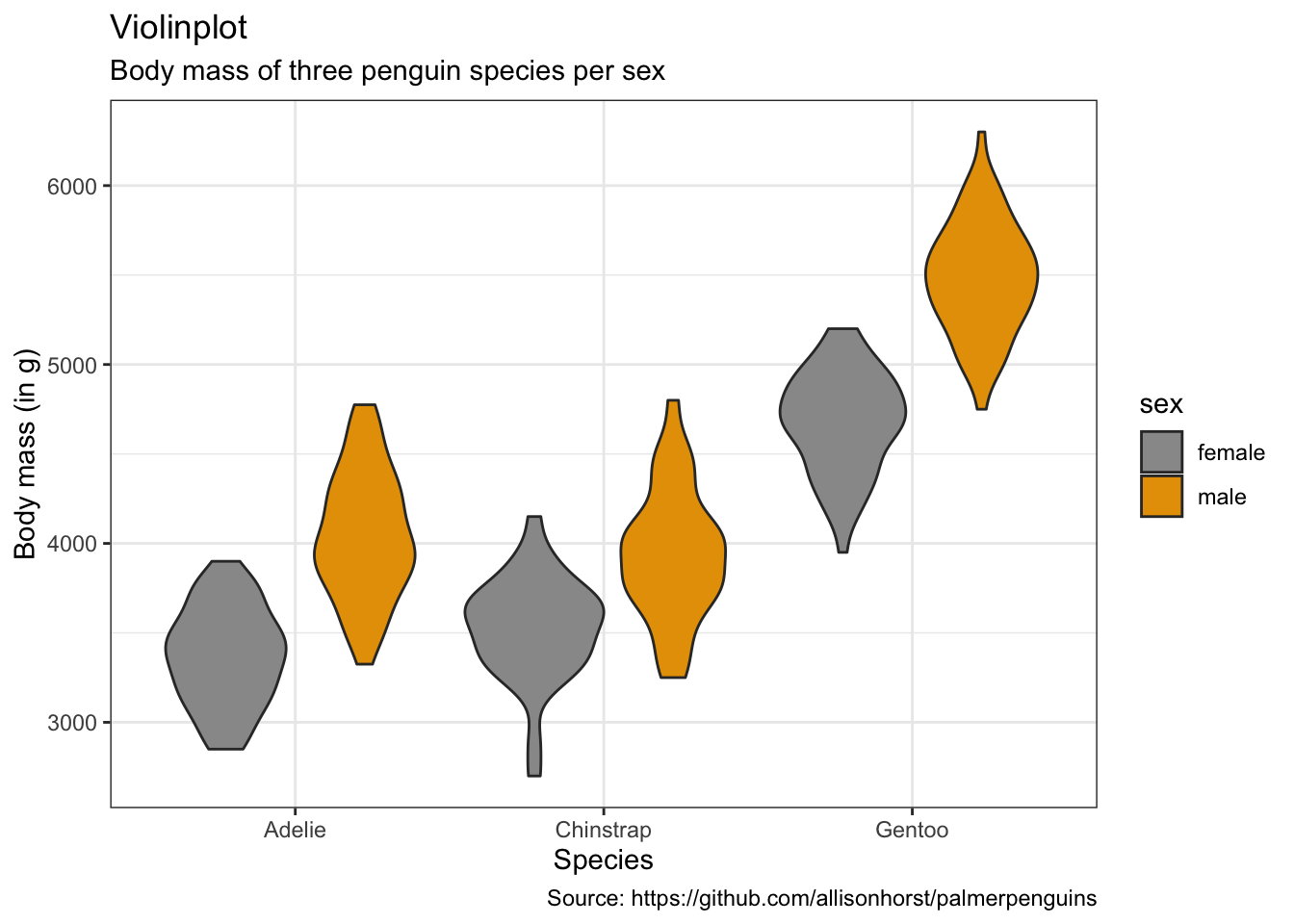• with dots (sina-plots)
``````penguins %>%
remove_missing() %>%
ggplot(aes(x = species, y = body_mass_g,
fill = sex, color = sex)) +
geom_dotplot(method = "dotdensity", alpha = 0.7,
binaxis = 'y', stackdir = 'center',
position = position_dodge(1)) +
labs(x = "Species",
y = "Body mass (in g)",
title = "Violinplot with points (dotplot)",
subtitle = "Body mass of three penguin species per sex",
caption = "Source: https://github.com/allisonhorst/palmerpenguins")``````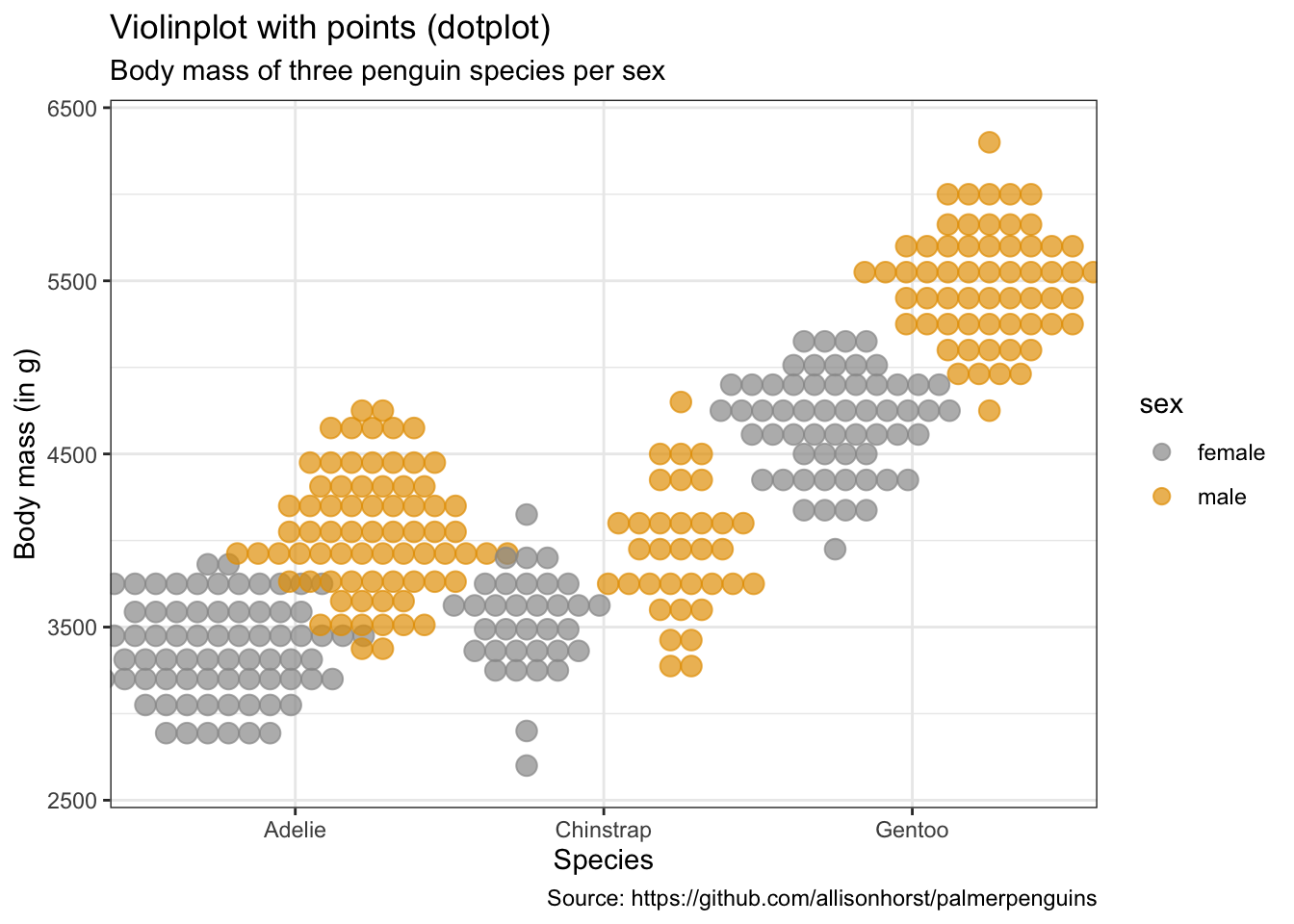### Piecharts

``````penguins %>%
remove_missing() %>%
group_by(species, sex) %>%
summarise(n = n()) %>%
mutate(freq = n / sum(n),
percentage = freq * 100) %>%
ggplot(aes(x = "", y = percentage,
fill = sex)) +
facet_wrap(vars(species), nrow = 1) +
geom_bar(stat = "identity", alpha = 0.8) +
coord_polar("y", start = 0) +
labs(x = "",
y = "Percentage",
title = "Piechart",
subtitle = "Percentage of male v. female penguins per species in study",
caption = "Source: https://github.com/allisonhorst/palmerpenguins")``````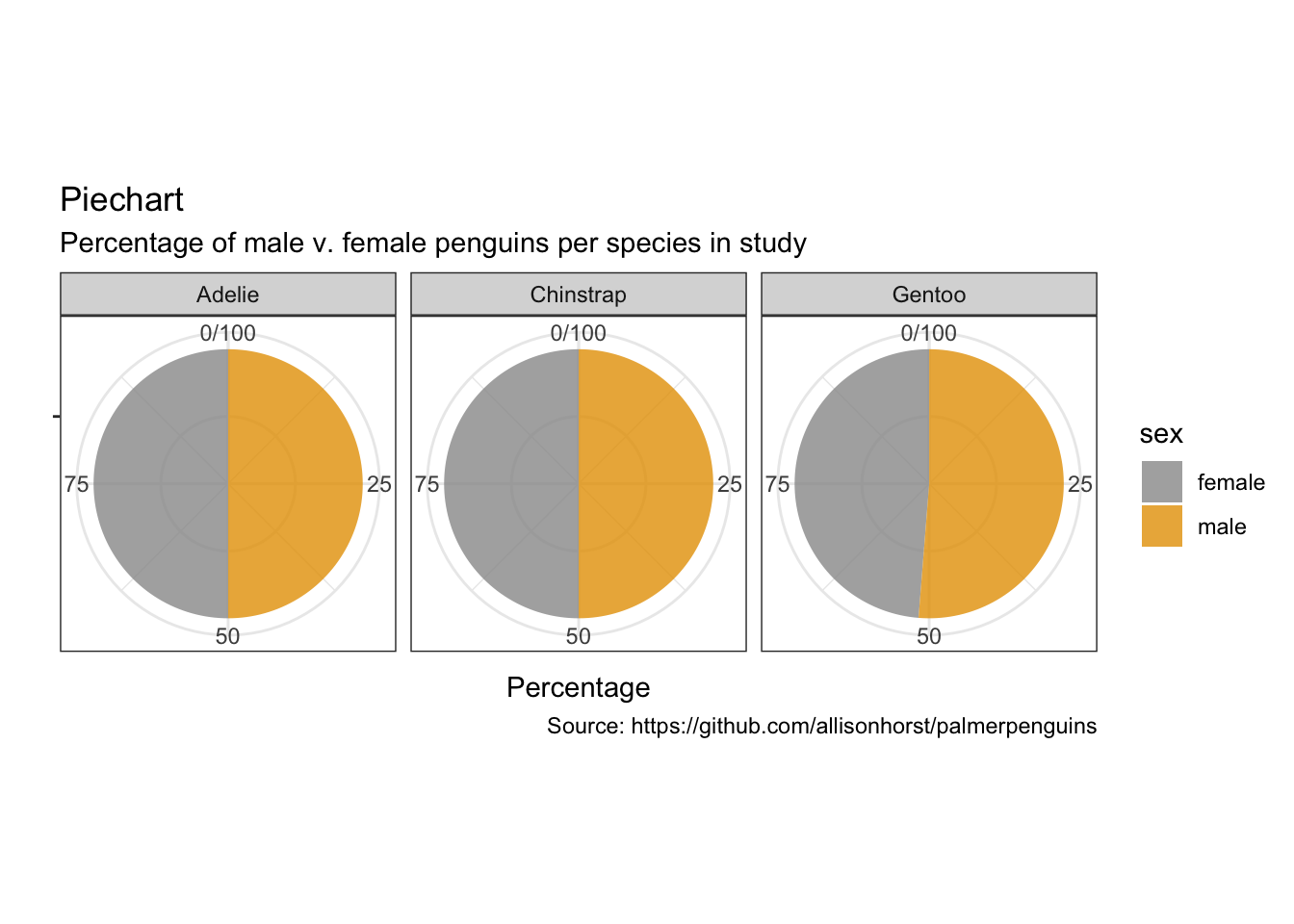### Alluvial charts

``````as.data.frame(UCBAdmissions) %>%
ggplot(aes(y = Freq, axis1 = Gender, axis2 = Dept)) +
geom_alluvium(aes(fill = Admit), width = 1/12) +
geom_stratum(width = 1/12, fill = "black", color = "grey") +
geom_label(stat = "stratum", aes(label = after_stat(stratum))) +
scale_x_discrete(limits = c("Gender", "Dept"), expand = c(.05, .05)) +
labs(x = "",
y = "Frequency",
title = "Alluvial chart",
subtitle = "UC Berkeley admissions and rejections, by sex and department",
caption = "Source: Bickel et al. (1975)\nSex bias in graduate admissions: Data from Berkeley. Science, 187, 398–403.")``````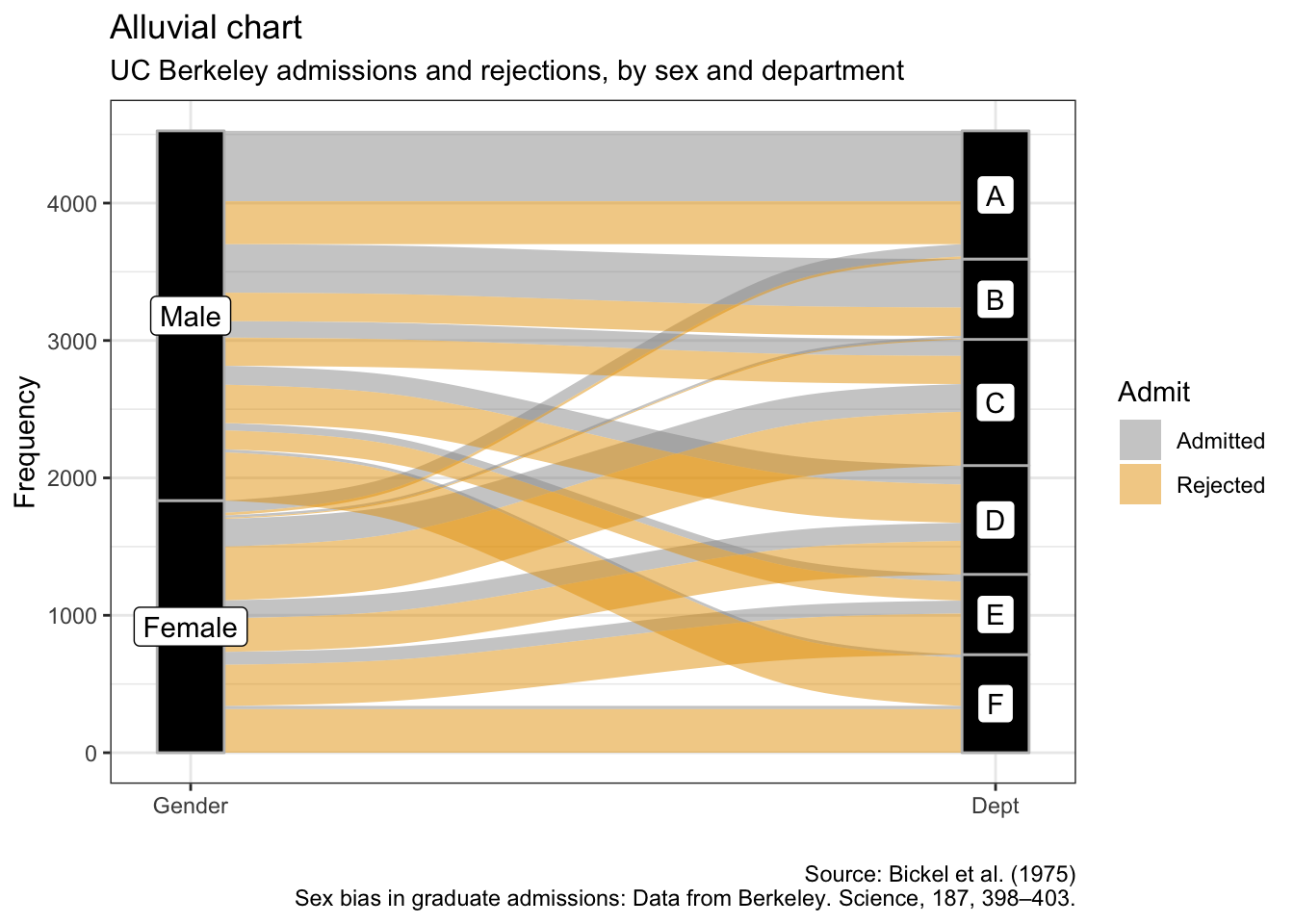### Treemaps

``````as.data.frame(UCBAdmissions) %>%
summarise(sum_freq = sum(Freq)) %>%
ggplot(aes(area = sum_freq, fill = sum_freq, label = Gender,
geom_treemap() +
geom_treemap_subgroup_border() +
geom_treemap_subgroup_text(place = "centre", grow = T, alpha = 0.5, colour =
"black", fontface = "italic", min.size = 0) +
geom_treemap_text(colour = "white", place = "centre", reflow = T) +
scale_fill_gradient2(low = "#999999", high = "#E69F00", mid = "white", midpoint = 1000, space = "Lab",
name = "Sum of\nfrequencies") +
labs(x = "",
y = "",
title = "Treemap",
subtitle = "UC Berkeley admissions and rejections by sex",
caption = "Source: Bickel et al. (1975)\nSex bias in graduate admissions: Data from Berkeley. Science, 187, 398–403.")``````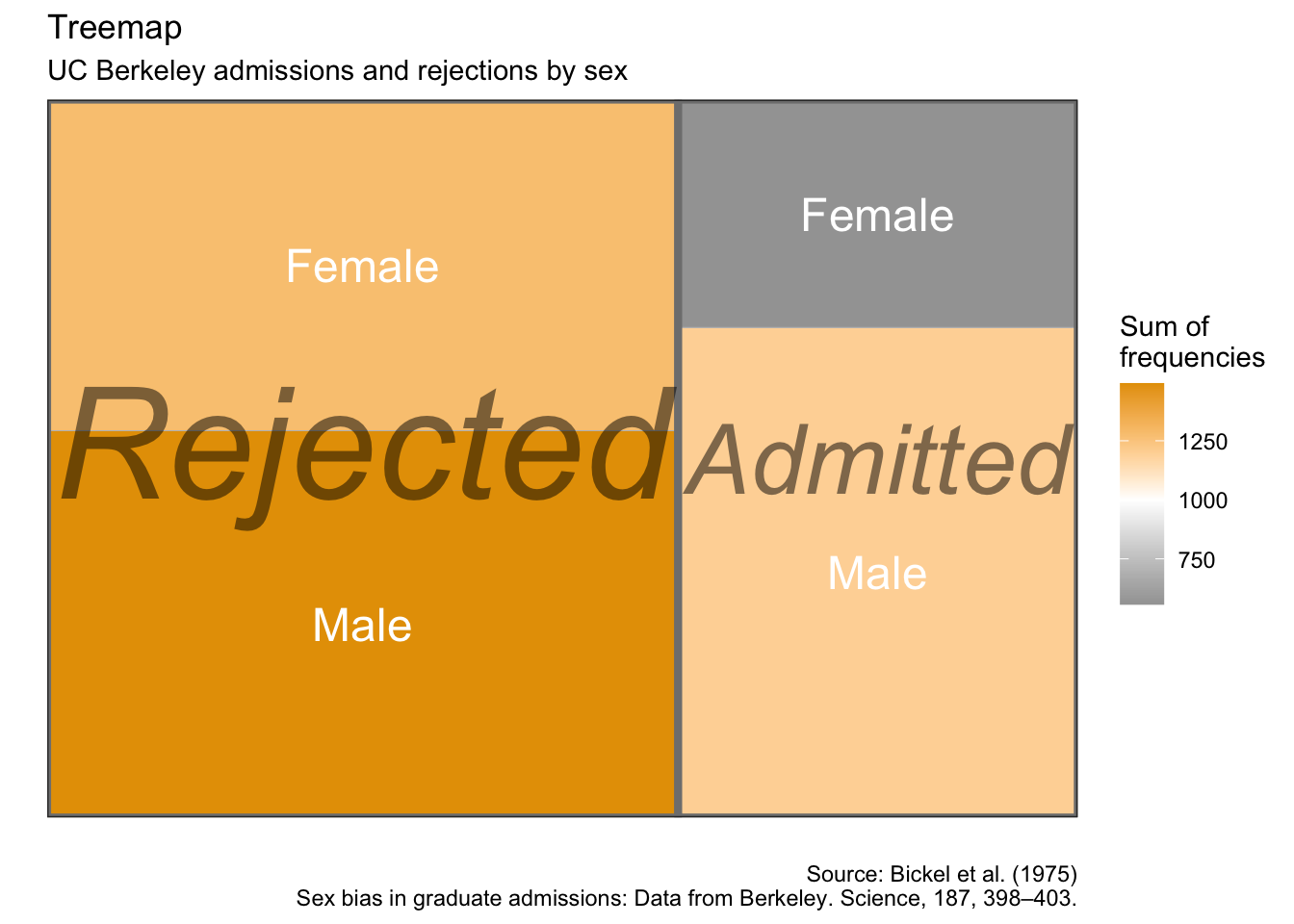### Dumbbell plots

``````penguins %>%
remove_missing() %>%
group_by(year, species, sex) %>%
summarise(mean_bmg = mean(body_mass_g)) %>%
mutate(species_sex = paste(species, sex, sep = "_"),
year = paste0("year_", year)) %>%
ggplot(aes(x = year_2007, xend = year_2009,
y = reorder(species_sex, year_2009))) +
geom_dumbbell(color = "#999999",
size_x = 3,
size_xend = 3,
#Note: there is no US:'color' for UK:'colour'
# in geom_dumbbel unlike standard geoms in ggplot()
colour_x = "#999999",
colour_xend = "#E69F00") +
labs(x = "Body mass (g)",
y = "Species & sex",
title = "Dumbbell plot",
subtitle = "Penguin's change in body mass from 2007 to 2009",
caption = "Source: https://github.com/allisonhorst/palmerpenguins")``````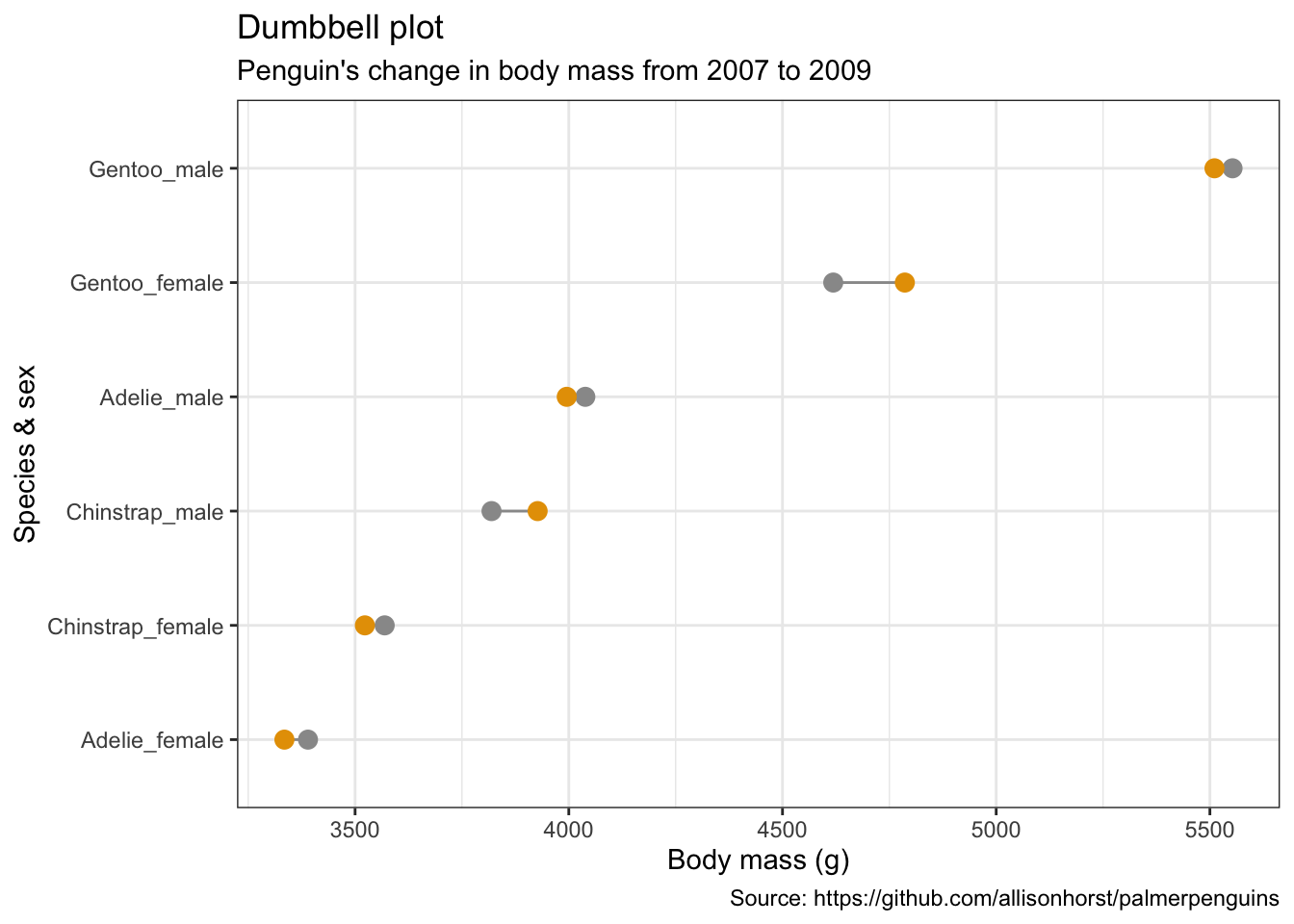### Slope charts

``````penguins %>%
remove_missing() %>%
group_by(year, species, sex) %>%
summarise(mean_bmg = mean(body_mass_g)) %>%
ggplot(aes(x = year, y = mean_bmg, group = sex,
color = sex)) +
facet_wrap(vars(species), nrow = 3) +
geom_line(alpha = 0.6, size = 2) +
geom_point(alpha = 1, size = 3) +
scale_x_continuous(breaks=c(2007, 2008, 2009)) +
labs(x = "Year",
y = "Body mass (g)",
color = "Sex",
title = "Slope chart",
subtitle = "Penguin's change in body mass from 2007 to 2009",
caption = "Source: https://github.com/allisonhorst/palmerpenguins")``````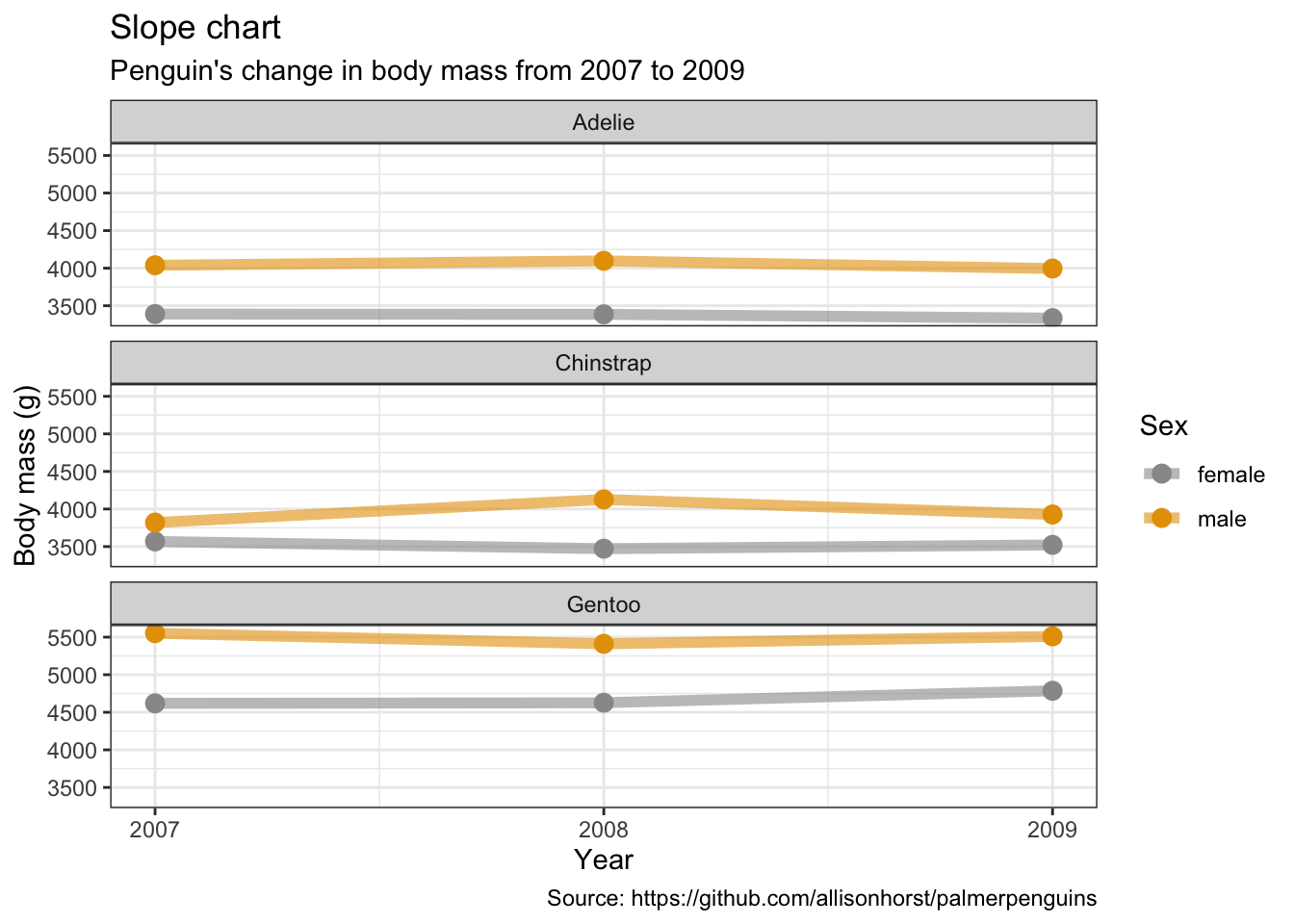### Stacked area charts

``````penguins %>%
remove_missing() %>%
group_by(year, species, sex) %>%
summarise(mean_bmg = mean(body_mass_g)) %>%
ggplot(aes(x = year, y = mean_bmg, fill = sex)) +
facet_wrap(vars(species), nrow = 3) +
geom_area(alpha = 0.6, size=.5, color = "white") +
scale_x_continuous(breaks=c(2007, 2008, 2009)) +
labs(x = "Year",
y = "Mean body mass (g)",
color = "Sex",
title = "Stacked area chart",
subtitle = "Penguin's change in body mass from 2007 to 2009",
caption = "Source: https://github.com/allisonhorst/palmerpenguins")``````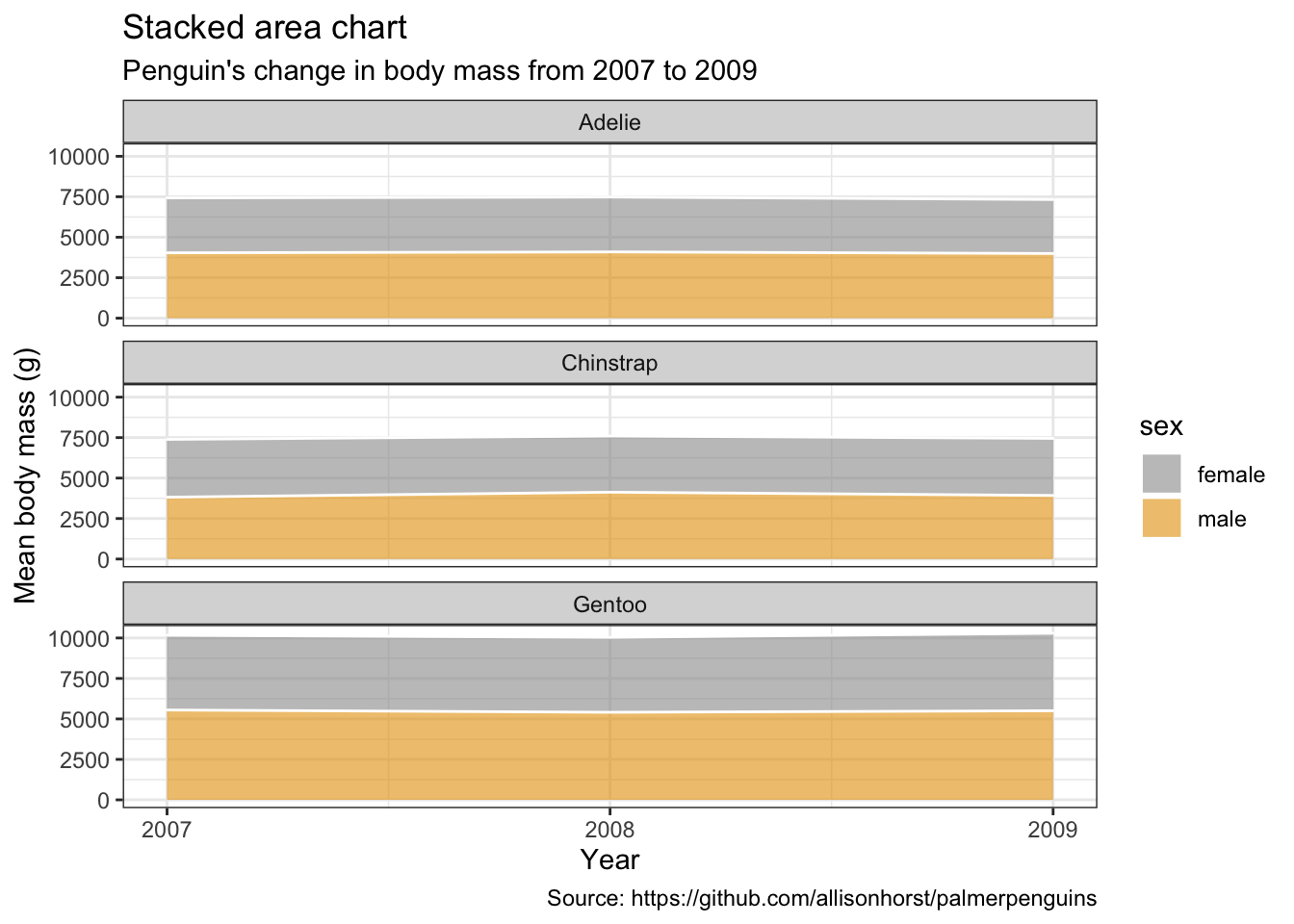### Lolliplot chart

``````penguins %>%
remove_missing() %>%
group_by(year, species, sex) %>%
summarise(mean_bmg = mean(body_mass_g)) %>%
mutate(species_sex = paste(species, sex, sep = "_"),
year = paste0("year_", year)) %>%
ggplot() +
geom_segment(aes(x = reorder(species_sex, -year_2009), xend = reorder(species_sex, -year_2009),
y = 0, yend = year_2009),
color = "#999999", size = 1) +
geom_point(aes(x = reorder(species_sex, -year_2009), y = year_2009),
size = 4, color = "#E69F00") +
coord_flip() +
labs(x = "Species & sex",
y = "Body mass (g)",
title = "Lollipop chart",
subtitle = "Penguin's body mass in 2009",
caption = "Source: https://github.com/allisonhorst/palmerpenguins")``````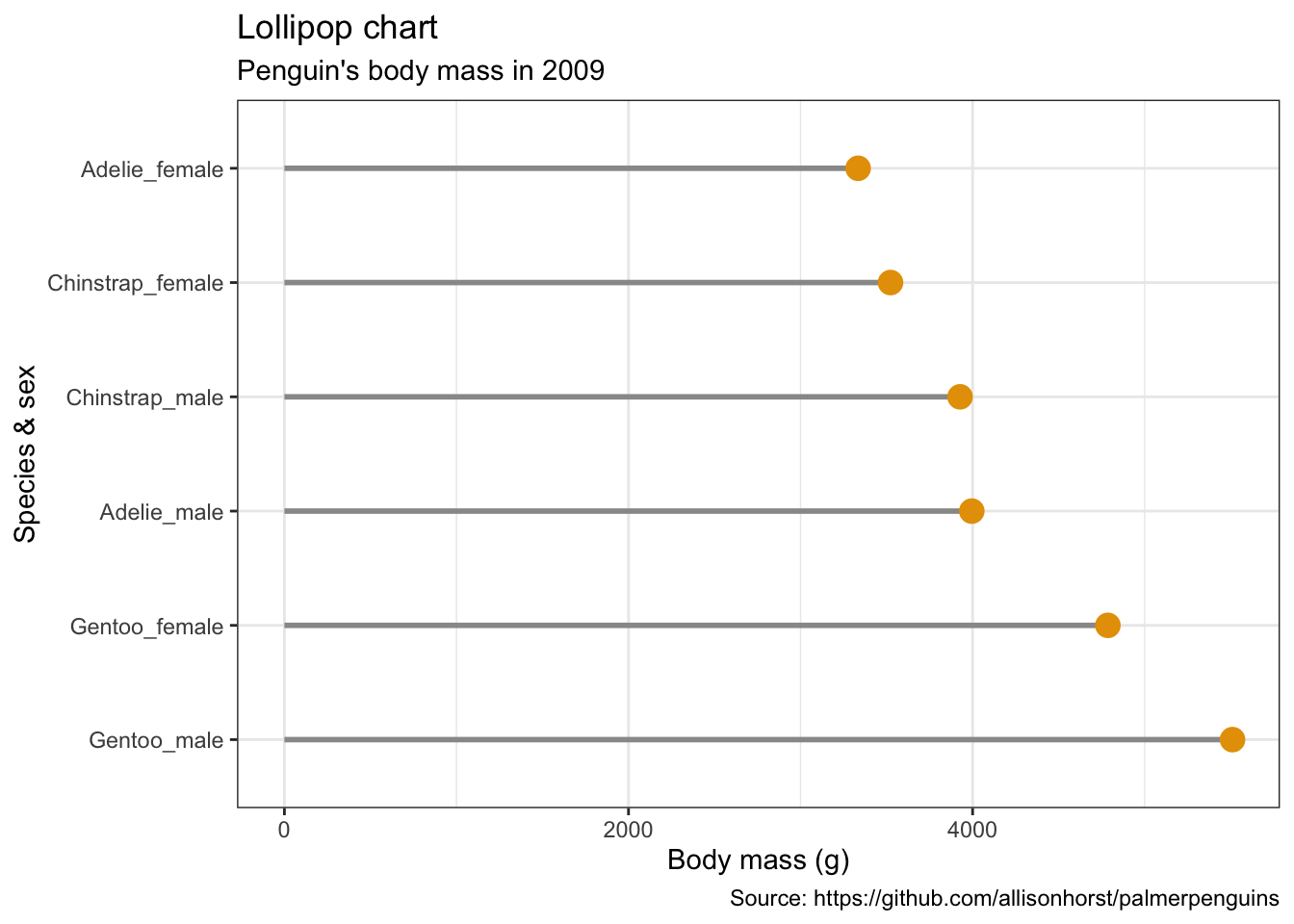### Dendrograms

``````library(ggdendro)
library(dendextend)``````
``````penguins_hist <- penguins %>%
filter(sex == "male") %>%
select(species, bill_length_mm, bill_depth_mm, flipper_length_mm, body_mass_g) %>%
group_by(species) %>%
sample_n(10) %>%
as.data.frame()
rownames(penguins_hist) <- paste(penguins_hist\$species, seq_len(nrow(penguins_hist)), sep = "_")

penguins_hist <- penguins_hist %>%
select(-species) %>%
remove_missing()``````
``````#hc <- hclust(dist(penguins_hist, method = "euclidean"), method = "ward.D2")
#ggdendrogram(hc)

# Create a dendrogram and plot it
penguins_hist %>%
scale %>%
dist(method = "euclidean") %>%
hclust(method = "ward.D2") %>%
as.dendrogram``````
``## 'dendrogram' with 2 branches and 30 members total, at height 11.94105``

### Waterfall charts

``library(waterfall)``
``````jaquith %>%
arrange(score) %>%
add_row(factor = "Total", score = sum(jaquith\$score)) %>%
mutate(factor = factor(factor, levels = factor),
id = seq_along(score)) %>%
mutate(end = cumsum(score),
start = c(0, end[-length(end)]),
start = c(start[-length(start)], 0),
end = c(end[-length(end)], score[length(score)]),
gr_col = ifelse(factor == "Total", "Total", "Part")) %>%
ggplot(aes(x = factor, fill = gr_col)) +
geom_rect(aes(x = factor,
xmin = id - 0.45, xmax = id + 0.45,
ymin = end, ymax = start)) +
theme(axis.text.x = element_text(angle = 60, vjust = 1, hjust = 1),
legend.position = "none") +
labs(x = "",
y = "Amount",
title = "Waterfall chart",
caption = "Andrew Jaquith, Security Metrics: Replacing Fear, Uncertainty, and Doubt\n(Boston: Addison-Wesley Professional, 2007), 170-171.")``````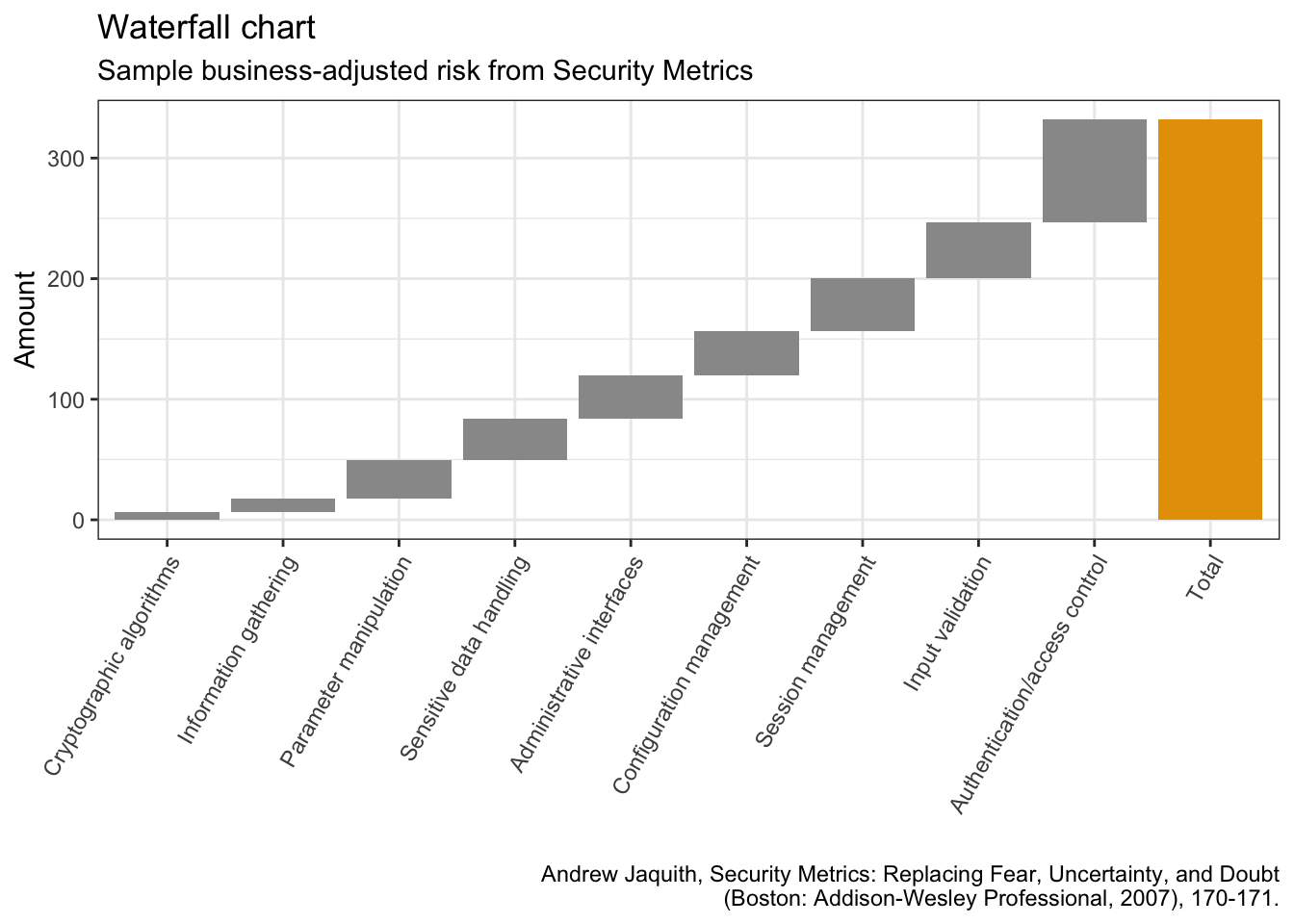### Biplots

``library(ggfortify)``
``````penguins_prep <- penguins %>%
remove_missing() %>%
select(bill_length_mm:body_mass_g)

penguins_pca <- penguins_prep %>%
prcomp(scale. = TRUE)``````
``````penguins_km <- penguins_prep %>%
kmeans(3)``````
``````autoplot(penguins_pca,
data = penguins %>% remove_missing(),
colour = 'species',
shape = 'species',
scale_color_manual(values = cbp1) +
scale_fill_manual(values = cbp1) +
theme_bw() +
labs(
title = "Biplot PCA",
caption = "Source: https://github.com/allisonhorst/palmerpenguins")``````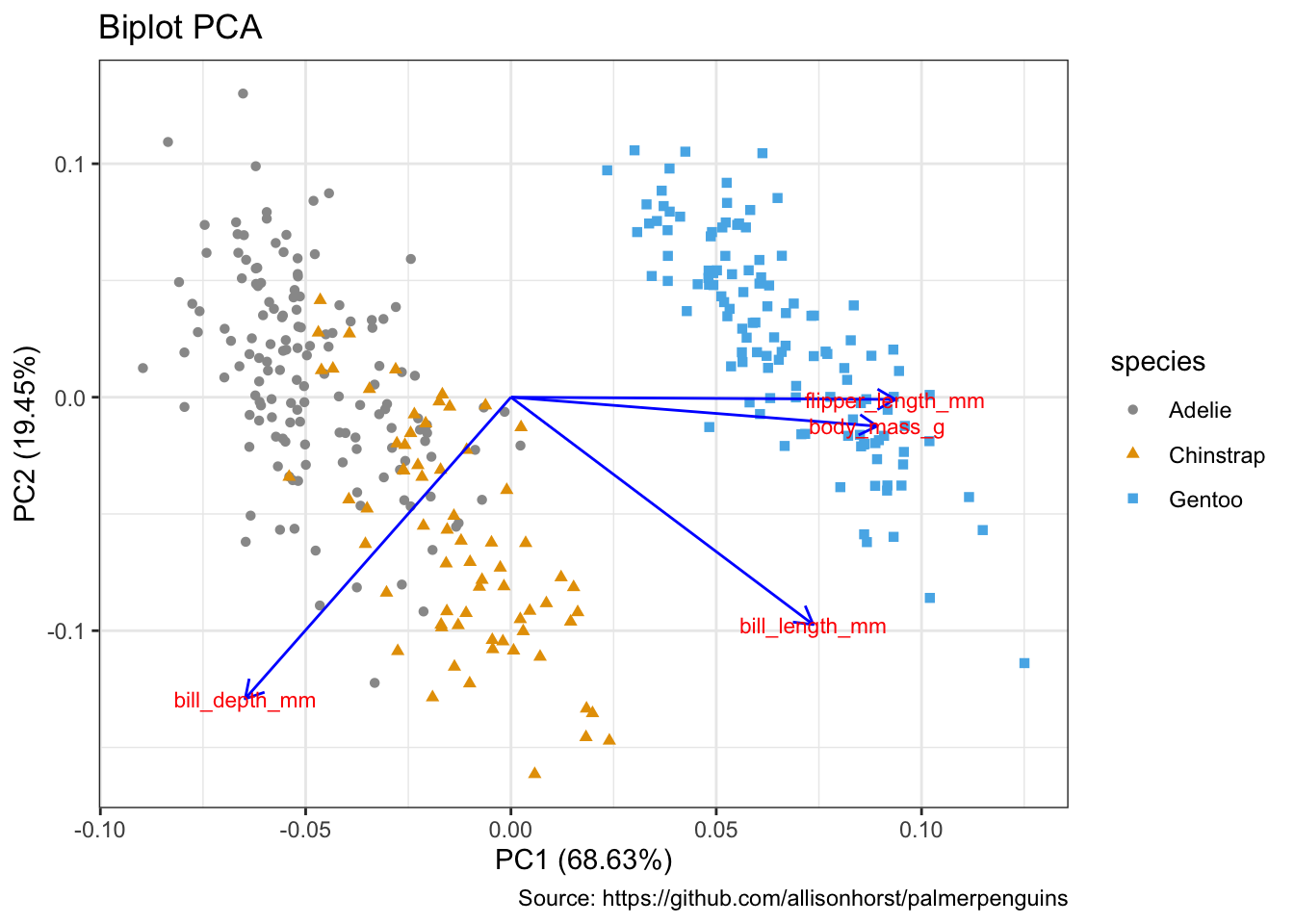``````autoplot(penguins_km,
data = penguins %>% remove_missing(),
colour = 'species',
shape = 'species',
frame = TRUE, frame.type = 'norm') +
scale_color_manual(values = cbp1) +
scale_fill_manual(values = cbp1) +
theme_bw() +
labs(
title = "Biplot k-Means clustering",
caption = "Source: https://github.com/allisonhorst/palmerpenguins")``````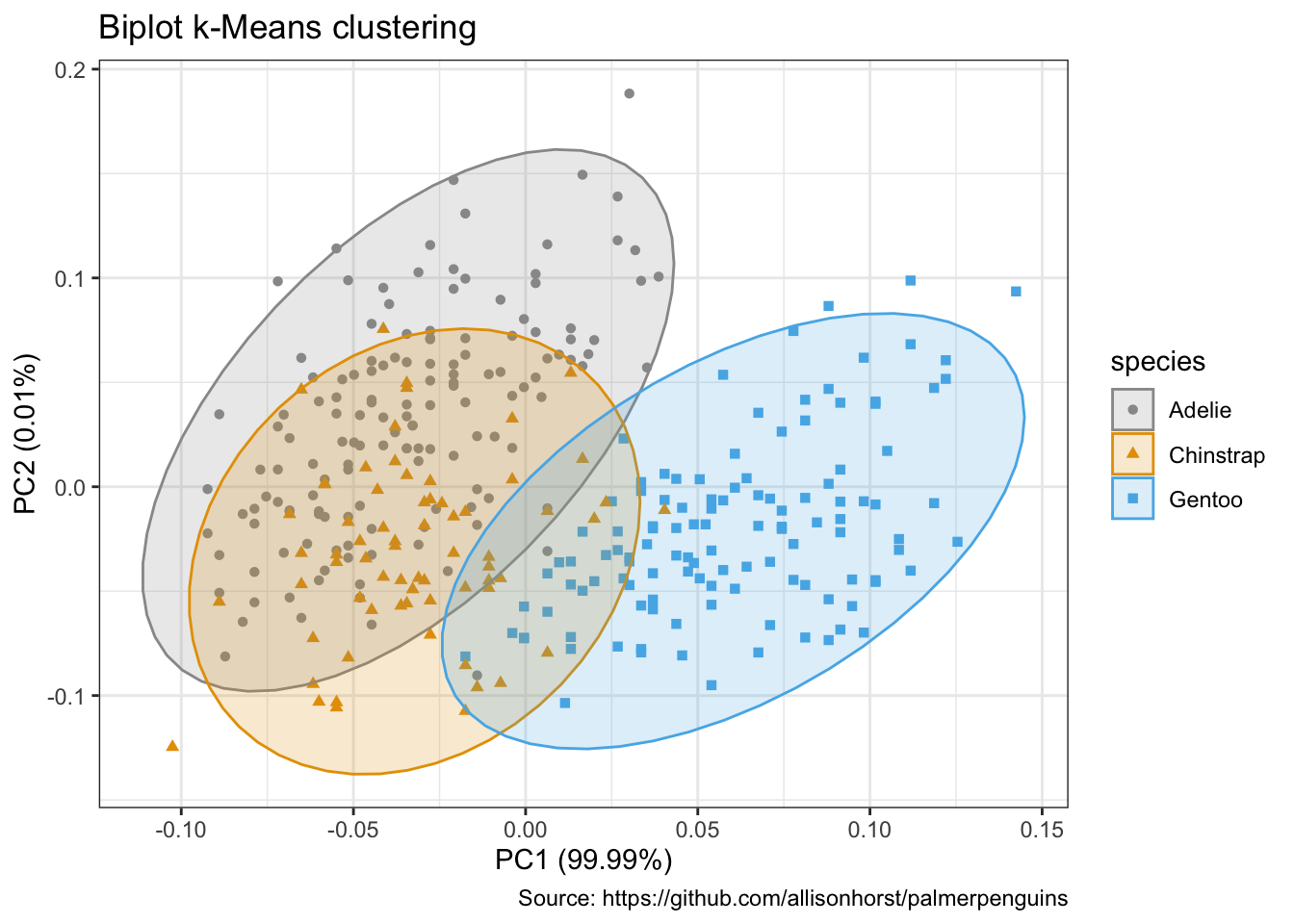### Radar charts, aka star chart, aka spider plot

https://www.data-to-viz.com/caveat/spider.html

``library(ggiraphExtra)``
``````penguins %>%
remove_missing() %>%
select(-island, -year) %>%
ggRadar(aes(x = c(bill_length_mm, bill_depth_mm, flipper_length_mm, body_mass_g),
group = species,
colour = sex, facet = sex),
rescale = TRUE,
size = 1, interactive = FALSE,
use.label = TRUE) +
scale_color_manual(values = cbp1) +
scale_fill_manual(values = cbp1) +
theme_bw() +
scale_y_discrete(breaks = NULL) + # don't show ticks
labs(
subtitle = "Body mass of male & female penguins per species",
caption = "Source: https://github.com/allisonhorst/palmerpenguins")``````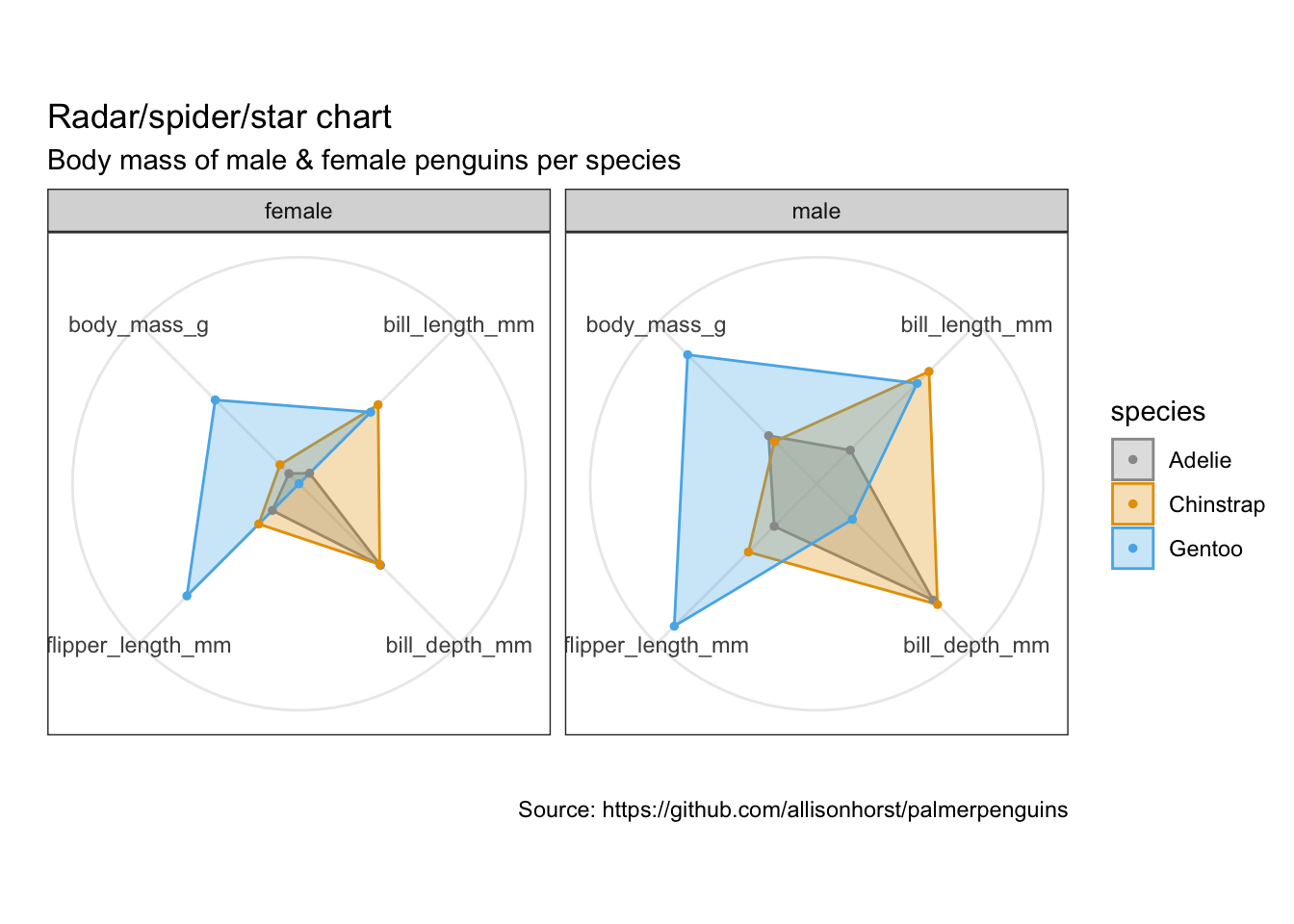``devtools::session_info()``
``````## ─ Session info ───────────────────────────────────────────────────────────────
##  setting  value
##  version  R version 4.0.2 (2020-06-22)
##  os       macOS Catalina 10.15.7
##  system   x86_64, darwin17.0
##  ui       X11
##  language (EN)
##  collate  en_US.UTF-8
##  ctype    en_US.UTF-8
##  tz       Europe/Berlin
##  date     2020-10-20
##
## ─ Packages ───────────────────────────────────────────────────────────────────
##  package        * version date       lib source
##  ash              1.0-15  2015-09-01  CRAN (R 4.0.2)
##  assertthat       0.2.1   2019-03-21  CRAN (R 4.0.0)
##  backports        1.1.10  2020-09-15  CRAN (R 4.0.2)
##  blob             1.2.1   2020-01-20  CRAN (R 4.0.2)
##  blogdown         0.20.1  2020-09-09  Github (rstudio/blogdown@d96fe78)
##  bookdown         0.20    2020-06-23  CRAN (R 4.0.2)
##  broom            0.7.0   2020-07-09  CRAN (R 4.0.2)
##  callr            3.4.4   2020-09-07  CRAN (R 4.0.2)
##  cellranger       1.1.0   2016-07-27  CRAN (R 4.0.0)
##  cli              2.0.2   2020-02-28  CRAN (R 4.0.0)
##  colorspace       1.4-1   2019-03-18  CRAN (R 4.0.0)
##  crayon           1.3.4   2017-09-16  CRAN (R 4.0.0)
##  DBI              1.1.0   2019-12-15  CRAN (R 4.0.0)
##  dbplyr           1.4.4   2020-05-27  CRAN (R 4.0.2)
##  dendextend     * 1.14.0  2020-08-26  CRAN (R 4.0.2)
##  desc             1.2.0   2018-05-01  CRAN (R 4.0.0)
##  devtools         2.3.2   2020-09-18  CRAN (R 4.0.2)
##  digest           0.6.25  2020-02-23  CRAN (R 4.0.0)
##  dplyr          * 1.0.2   2020-08-18  CRAN (R 4.0.2)
##  ellipsis         0.3.1   2020-05-15  CRAN (R 4.0.0)
##  evaluate         0.14    2019-05-28  CRAN (R 4.0.1)
##  extrafont        0.17    2014-12-08  CRAN (R 4.0.2)
##  extrafontdb      1.0     2012-06-11  CRAN (R 4.0.2)
##  fansi            0.4.1   2020-01-08  CRAN (R 4.0.0)
##  farver           2.0.3   2020-01-16  CRAN (R 4.0.0)
##  fastmap          1.0.1   2019-10-08  CRAN (R 4.0.0)
##  forcats        * 0.5.0   2020-03-01  CRAN (R 4.0.0)
##  fs               1.5.0   2020-07-31  CRAN (R 4.0.2)
##  gdtools          0.2.2   2020-04-03  CRAN (R 4.0.2)
##  generics         0.0.2   2018-11-29  CRAN (R 4.0.0)
##  ggalluvial     * 0.12.2  2020-08-30  CRAN (R 4.0.2)
##  ggalt          * 0.4.0   2017-02-15  CRAN (R 4.0.2)
##  ggdendro       * 0.1.22  2020-09-13  CRAN (R 4.0.2)
##  ggExtra        * 0.9     2019-08-27  CRAN (R 4.0.2)
##  ggfittext        0.9.0   2020-06-14  CRAN (R 4.0.2)
##  ggfortify      * 0.4.10  2020-04-26  CRAN (R 4.0.2)
##  ggiraph          0.7.8   2020-07-01  CRAN (R 4.0.2)
##  ggiraphExtra   * 0.2.9   2018-07-22  CRAN (R 4.0.2)
##  ggplot2        * 3.3.2   2020-06-19  CRAN (R 4.0.2)
##  glue             1.4.2   2020-08-27  CRAN (R 4.0.2)
##  gridExtra        2.3     2017-09-09  CRAN (R 4.0.2)
##  gtable           0.3.0   2019-03-25  CRAN (R 4.0.0)
##  haven            2.3.1   2020-06-01  CRAN (R 4.0.2)
##  hms              0.5.3   2020-01-08  CRAN (R 4.0.0)
##  htmltools        0.5.0   2020-06-16  CRAN (R 4.0.2)
##  htmlwidgets      1.5.1   2019-10-08  CRAN (R 4.0.0)
##  httpuv           1.5.4   2020-06-06  CRAN (R 4.0.2)
##  httr             1.4.2   2020-07-20  CRAN (R 4.0.2)
##  insight          0.9.6   2020-09-20  CRAN (R 4.0.2)
##  jsonlite         1.7.1   2020-09-07  CRAN (R 4.0.2)
##  KernSmooth       2.23-17 2020-04-26  CRAN (R 4.0.2)
##  knitr            1.30    2020-09-22  CRAN (R 4.0.2)
##  labeling         0.3     2014-08-23  CRAN (R 4.0.0)
##  later            1.1.0.1 2020-06-05  CRAN (R 4.0.2)
##  lattice        * 0.20-41 2020-04-02  CRAN (R 4.0.2)
##  lifecycle        0.2.0   2020-03-06  CRAN (R 4.0.0)
##  lubridate        1.7.9   2020-06-08  CRAN (R 4.0.2)
##  magrittr         1.5     2014-11-22  CRAN (R 4.0.0)
##  maps             3.3.0   2018-04-03  CRAN (R 4.0.2)
##  MASS             7.3-53  2020-09-09  CRAN (R 4.0.2)
##  Matrix           1.2-18  2019-11-27  CRAN (R 4.0.2)
##  memoise          1.1.0   2017-04-21  CRAN (R 4.0.0)
##  mgcv             1.8-33  2020-08-27  CRAN (R 4.0.2)
##  mime             0.9     2020-02-04  CRAN (R 4.0.0)
##  miniUI           0.1.1.1 2018-05-18  CRAN (R 4.0.0)
##  modelr           0.1.8   2020-05-19  CRAN (R 4.0.2)
##  munsell          0.5.0   2018-06-12  CRAN (R 4.0.0)
##  mycor            0.1.1   2018-04-10  CRAN (R 4.0.2)
##  nlme             3.1-149 2020-08-23  CRAN (R 4.0.2)
##  palmerpenguins * 0.1.0   2020-07-23  CRAN (R 4.0.2)
##  pillar           1.4.6   2020-07-10  CRAN (R 4.0.2)
##  pkgbuild         1.1.0   2020-07-13  CRAN (R 4.0.2)
##  pkgconfig        2.0.3   2019-09-22  CRAN (R 4.0.0)
##  pkgload          1.1.0   2020-05-29  CRAN (R 4.0.2)
##  plotrix        * 3.7-8   2020-04-16  CRAN (R 4.0.2)
##  plyr             1.8.6   2020-03-03  CRAN (R 4.0.0)
##  ppcor            1.1     2015-12-03  CRAN (R 4.0.2)
##  prettyunits      1.1.1   2020-01-24  CRAN (R 4.0.0)
##  processx         3.4.4   2020-09-03  CRAN (R 4.0.2)
##  proj4            1.0-10  2020-03-02  CRAN (R 4.0.1)
##  promises         1.1.1   2020-06-09  CRAN (R 4.0.2)
##  ps               1.3.4   2020-08-11  CRAN (R 4.0.2)
##  purrr          * 0.3.4   2020-04-17  CRAN (R 4.0.0)
##  R6               2.4.1   2019-11-12  CRAN (R 4.0.0)
##  ragg           * 0.3.1   2020-07-03  CRAN (R 4.0.2)
##  RColorBrewer     1.1-2   2014-12-07  CRAN (R 4.0.0)
##  Rcpp             1.0.5   2020-07-06  CRAN (R 4.0.2)
##  readr          * 1.3.1   2018-12-21  CRAN (R 4.0.0)
##  readxl           1.3.1   2019-03-13  CRAN (R 4.0.0)
##  remotes          2.2.0   2020-07-21  CRAN (R 4.0.2)
##  reprex           0.3.0   2019-05-16  CRAN (R 4.0.0)
##  reshape2         1.4.4   2020-04-09  CRAN (R 4.0.0)
##  rlang            0.4.7   2020-07-09  CRAN (R 4.0.2)
##  rmarkdown        2.3     2020-06-18  CRAN (R 4.0.2)
##  rprojroot        1.3-2   2018-01-03  CRAN (R 4.0.0)
##  rstudioapi       0.11    2020-02-07  CRAN (R 4.0.0)
##  Rttf2pt1         1.3.8   2020-01-10  CRAN (R 4.0.2)
##  rvest            0.3.6   2020-07-25  CRAN (R 4.0.2)
##  scales           1.1.1   2020-05-11  CRAN (R 4.0.0)
##  sessioninfo      1.1.1   2018-11-05  CRAN (R 4.0.0)
##  shiny            1.5.0   2020-06-23  CRAN (R 4.0.2)
##  sjlabelled       1.1.7   2020-09-24  CRAN (R 4.0.2)
##  sjmisc           2.8.5   2020-05-28  CRAN (R 4.0.2)
##  stringi          1.5.3   2020-09-09  CRAN (R 4.0.2)
##  stringr        * 1.4.0   2019-02-10  CRAN (R 4.0.0)
##  systemfonts      0.3.2   2020-09-29  CRAN (R 4.0.2)
##  testthat         2.3.2   2020-03-02  CRAN (R 4.0.0)
##  tibble         * 3.0.3   2020-07-10  CRAN (R 4.0.2)
##  tidyr          * 1.1.2   2020-08-27  CRAN (R 4.0.2)
##  tidyselect       1.1.0   2020-05-11  CRAN (R 4.0.0)
##  tidyverse      * 1.3.0   2019-11-21  CRAN (R 4.0.0)
##  treemapify     * 2.5.3   2019-01-30  CRAN (R 4.0.2)
##  usethis          1.6.3   2020-09-17  CRAN (R 4.0.2)
##  utf8             1.1.4   2018-05-24  CRAN (R 4.0.0)
##  uuid             0.1-4   2020-02-26  CRAN (R 4.0.2)
##  vctrs            0.3.4   2020-08-29  CRAN (R 4.0.2)
##  viridis          0.5.1   2018-03-29  CRAN (R 4.0.2)
##  viridisLite      0.3.0   2018-02-01  CRAN (R 4.0.0)
##  waterfall      * 1.0.2   2016-04-03  CRAN (R 4.0.2)
##  withr            2.3.0   2020-09-22  CRAN (R 4.0.2)
##  xfun             0.18    2020-09-29  CRAN (R 4.0.2)
##  xml2             1.3.2   2020-04-23  CRAN (R 4.0.0)
##  xtable           1.8-4   2019-04-21  CRAN (R 4.0.0)
##  yaml             2.2.1   2020-02-01  CRAN (R 4.0.0)
##
##  /Library/Frameworks/R.framework/Versions/4.0/Resources/library``````